# Lower Columbia River Fish Population Indexing Analysis 2016

The suggested citation for this analytic report is:

Thorley, J.L. and Muir, C.D. (2017) Lower Columbia River Fish Population Indexing Analysis 2016. A Poisson Consulting Analysis Report. URL: https://www.poissonconsulting.ca/f/589633601.

## Background

In the mid 1990s BC Hydro began operating Hugh L. Keenleyside (HLK) Dam to reduce dewatering of Mountain Whitefish and Rainbow Trout eggs.

The primary goal of the Lower Columbia River Fish Population Indexing program is to answer two key management questions:

What are the abundance, growth rate, survival rate, body condition, age distribution, and spatial distribution of subadult and adult Whitefish, Rainbow Trout, and Walleye in the Lower Columbia River?

What is the effect of inter-annual variability in the Whitefish and Rainbow Trout flow regimes on the abundance, growth rate, survival rate, body condition, and spatial distribution of subadult and adult Whitefish, Rainbow Trout, and Walleye in the Lower Columbia River?

The inter-annual variability in the Whitefish and Rainbow Trout flow regimes was quantified in terms of the percent egg dewatering as greater flow variability is associated with more egg stranding.

## Methods

### Data Preparation

The fish indexing data were provided by Okanagan Nation Alliance and Golder Associates in the form of an Access database. The discharge and temperature data were queried from a BC Hydro database maintained by Poisson Consulting. The Rainbow Trout egg dewatering estimates were provided by CLBMON-46 (Irvine, Baxter, and Thorley 2015) and the Mountain Whitefish egg stranding estimates by Golder Associates (2013).

The data were prepared for analysis using R version 3.4.0 (R Core Team 2017).

### Data Analysis

Hierarchical Bayesian models were fitted to the data using R version 3.4.0 (R Core Team 2017) and JAGS 4.2.0 (Plummer 2015) which interfaced with each other via the jmbr package. For additional information on hierarchical Bayesian modelling in the BUGS language, of which JAGS uses a dialect, the reader is referred to Kery and Schaub (2011, 41–44).

The one exception was the length-at-age analysis which used a maximum-likelihood (ML) model which was fitted to the data using TMB which interfaced with R via the tmbr package. An ML model was used because the equivalent Bayesian model was becoming prohibitively slow.

Unless indicated otherwise, the models used prior distributions that were vague in the sense that they did not affect the posterior distributions (Kery and Schaub 2011, 36). The posterior distributions were estimated from 2,000 Markov Chain Monte Carlo (MCMC) samples thinned from the second halves of three chains (Kery and Schaub 2011, 38–40). Model convergence was confirmed by ensuring that $$\hat{R} < 1.1$$ (Kery and Schaub 2011, 40) for each of the monitored parameters in the model(Kery and Schaub 2011, 61). Where relevant, model adequacy was confirmed by examination of residual plots.

The posterior distributions of the fixed (Kery and Schaub 2011, 75) parameters are summarised in terms of the point estimate, standard deviation (sd), the z-score, lower and upper 95% confidence/credible limits (CLs) and the p-value (Kery and Schaub 2011, 37, 42). The estimate is the median (50th percentile) of the MCMC samples, the z-score is $$\mathrm{mean}/\mathrm{sd}$$ and the 95% CLs are the 2.5th and 97.5th percentiles. A p-value of 0.05 indicates that the lower or upper 95% CL is 0.

Variable selection was achieved by dropping fixed (Kery and Schaub 2011, 77–82) variables with two-sided p-values $$\geq$$ 0.05 (Kery and Schaub 2011, 37, 42) and random variables with percent relative errors $$\geq$$ 80%.

The results are displayed graphically by plotting the modeled relationships between particular variables and the response with 95% credible intervals (CIs) with the remaining variables held constant. In general, continuous and discrete fixed variables are held constant at their mean and first level values respectively while random variables are held constant at their typical values (expected values of the underlying hyperdistributions) (Kery and Schaub 2011, 77–82). Where informative the influence of particular variables is expressed in terms of the effect size (i.e., percent change in the response variable) with 95% CIs (Bradford, Korman, and Higgins 2005).

### Model Descriptions

#### Condition

The expected weight of fish of a given length were estimated from the data using a mass-length model (He et al. 2008). Key assumptions of the condition model include:

• The expected weight is allowed to vary with length and date.
• The expected weight is allowed to vary randomly with year.
• The relationship between weight and length is allowed to vary with date.
• The relationship between weight and length is allowed to vary randomly with year.
• The residual variation in weight is log-normally distributed.

Only previously untagged fish were included in models to avoid potential effects of tagging on body condition. Preliminary analyses indicated that the annual variation in weight was not correlated with the annual variation in the relationship between weight and length.

#### Growth

Annual growth of fish were estimated from the inter-annual recaptures using the Fabens method (Fabens 1965) for estimating the von Bertalanffy growth curve (von Bertalanffy 1938). This curve is based on the premise that:

$\frac{\text{d}L}{\text{d}t} = k (L_{\infty} - L)$

where $$L$$ is the length of the individual, $$k$$ is the growth coefficient and $$L_{\infty}$$ is the maximum length.

Integrating the above equation gives:

$L_t = L_{\infty} (1 - e^{-k(t - t_0)})$

where $$L_t$$ is the length at time $$t$$ and $$t_0$$ is the time at which the individual would have had zero length.

The Fabens form allows

$L_r = L_c + (L_{\infty} - L_c) (1 - e^{-kT})$

where $$L_r$$ is the length at recapture, $$L_c$$ is the length at capture and $$T$$ is the time between capture and recapture.

Key assumptions of the growth model include:

• The mean maximum length $$L_{\infty}$$ is constant.
• The growth coefficient $$k$$ is allowed to vary randomly with year.
• The residual variation in growth is normally distributed.

#### Length-At-Age

The expected length-at-age of Mountain Whitefish and Rainbow Trout were estimated from annual length-frequency distributions using a finite mixture distribution model (Macdonald and Pitcher 1979). Key assumptions of the length-at-age model include:

• There are three distinguishable age-classes for each species: Age-0, Age-1 and Age-2+.
• The proportion of fish in each age-class is allowed to vary with year.
• The expected length increases with age-class.
• The expected length is allowed to vary with year within age-class.
• The expected length is allowed to vary as a linear function of date.
• The relationship between length and date is allowed to vary with age-class.
• The residual variation in log-tranformed length is normally distributed.
• The standard deviation of this normal distribution varies with age-class.

The model was used to estimate the cutoffs between age-classes by year and date within year to account for growth. For the purposes of estimating other population parameters by age-class, Age-0 individuals were classified as fry, Age-1 individuals were classified as subadult, and Age-2+ individuals were classified as adult. Walleye could not be separated by life stage due to a lack of discrete modes in the length-frequency distributions for this species. Consequently, all captured Walleye were considered to be adults.

The results include plots of the age-class density for each year by length as predicted by the length-at-age model. Density is a measure of relative frequency for continuous values.

#### Observer Length Correction

The bias (accuracy) and error (precision) in observer’s fish length estimates were quantified using a model with a categorical distribution which compared the proportions of fish in different length-classes for each observer to the equivalent proportions for the measured fish. Key assumptions of the observer length correction model include:

• The proportion of fish in each length-class is allowed to vary with year.
• The expected length bias is allowed to vary with observer.
• The expected length error is allowed to vary with observer.
• The expected length bias and error for a given observer does not vary by year.
• The residual variation in length is normally distributed.

The observed fish lengths were corrected for the estimated length biases before being classified as fry, subadult and adult based on the length-at-age cutoffs.

#### Survival

The annual adult survival rate was estimated by fitting a Cormack-Jolly-Seber model (Kery and Schaub 2011, 220–31) to inter-annual recaptures of adults.

Key assumptions of the survival model include:

• Survival varies randomly with year.
• The encounter probability for adults is allowed to vary with the total bank length sampled.

#### Site Fidelity

The extent to which sites are closed, i.e., fish remain at the same site between sessions, was evaluated with a logistic ANCOVA (Kery 2010). The model estimates the probability that intra-annual recaptures were caught at the same site versus a different one. Key assumptions of the site fidelity model include:

• The expected site fidelity is allowed to vary with fish length.
• Observed site fidelity is Bernoulli distributed.

Length as a second-order polynomial was not found to be a significant predictor for site fidelity.

#### Capture Efficiency

The probability of capture was estimated using a recapture-based binomial model (Kery and Schaub 2011, 134–36, 384–88).

Key assumptions of the capture efficiency model include:

• The capture probability varies randomly by session within year.
• The probability of a marked fish remaining at a site is the estimated site fidelity.
• The number of recaptures is described by a binomial distribution.

#### Abundance

The abundance was estimated from the catch and bias-corrected observer count data using an overdispersed Poisson model (Kery and Schaub 2011, 55–56).

Key assumptions of the abundance model include:

• The capture efficiency is the point estimate from the capture efficiency model.
• The efficiency varies by visit type (catch or count).
• The lineal fish density varies randomly with site, year and site within year.
• The overdispersion varies by visit type (count or catch).
• The catches and counts are described by a gamma-Poisson distribution.

#### Stock-Recruitment

The relationship between the adults and the resultant number of age-1 subadults was estimated using a Beverton-Holt stock-recruitment model (Walters and Martell 2004):

$R = \frac{\alpha \cdot S}{1 + \beta \cdot S} \quad,$

where $$S$$ is the adults (stock), $$R$$ is the subadults (recruits), $$\alpha$$ is the recruits per spawner at low density and $$\beta$$ determines the density-dependence.

Key assumptions of the stock-recruitment model include:

• The prior for $$\log(\alpha)$$ is a truncated normal distribution from $$\log(1)$$ to $$\log(5)$$.
• The recruits per spawner varies with the proportional egg loss.
• The residual variation in the number of recruits is log-normally distributed.

The carrying capacity $$K$$ is given by the relationship:

$K = \frac{\alpha}{\beta} \quad.$

#### Scale Age

The age of Mountain Whitefish was estimated from scales using an automated algorithm. The algorithm estimates the age of individual fish by finding annuli among the growth circuli in the scale. Specifically, annuli represent blocks of slow growth – short inter-circuli distance – during the winter. The algorithm takes scale growth between circuli, calculates a moving average growth rate, and then calculates a moving average of the rate of change in growth. For brevity, we refer to the “rate of change in growth” as “dGrowth”.Periods of rapid deceleration (dGrowth < 0) correspond to winter and mark an annulus. Specifically, we defined an annulus as a run of negative dGrowth values. Because of noise in the measurements, these runs had to be of a minimum length. We estimated the window size for calculating moving averages and this minimum length by tuning the algorithm to most accurately predict scale ages from recaptured fish of known age. During tuning, we found that a window size of seven circuli was best for calculating the moving average growth rate; a window size of 17 circuli was best for calculating dGrowth. Note that a larger window size leads to greater smoothing in the series. We estimated the minimum length for a run to be four. Once the algorithm was tuned, we applied to fish scales of unknown age.

Key assumptions of the scale age algorithm include:

• Growth decelerates in the fall/winter and accelerates in spring/summer
• The actual age is the year of capture minus the hatch year
• The scale age varies linearly with age.

#### Age-Ratios

The proportion of Age-1 Mountain Whitefish $$r^1_t$$ from a given spawn year $$t$$ is calculated from the number of Age-1 & Age-2 fish $$N^1_t$$ & $$N^2_t$$ respectively, which were lead or lagged so that all values were with respect to the spawn year:

$r^1_t = \frac{N^1_{t+2}}{N^1_{t+2} + N^2_{t+2}}$

As the number of Age-2 fish might be expected to be influenced by the percentage egg loss $$Q_t$$ three years prior, the predictor variable $$\Pi_t$$ used is:

$\Pi_t = \textrm{log}(Q_t/Q_{t-1})$

The ratio was logged to ensure it was symmetrical about zero (Tornqvist, Vartia, and Vartia 1985).

The relationship between $$r^1_t$$ and $$\Pi_t$$ was estimated using a hierarchical Bayesian logistic regression (Kery 2010) loss model.

Key assumptions of the final model include:

• The log odds of the proportion of Age-1 fish varies linearly with the log of the ratio of the percent egg losses.
• The numbers of Age-1 fish are extra-Binomially distributed.

The relationship between percent dewatering and subsequent recruitment is expected to depend on stock abundance (Subbey et al. 2014) which might be changing over the course of the study. Consequently, preliminary analyses allowed the slope of the regression line to change by year. However, year was not a significant predictor and was therefore removed from the final model. The effect of dewatering on Mountain Whitefish abundance was expressed in terms of the predicted percent change in Age-1 Mountain Whitefish abundance by egg loss in the spawn year relative to 10% egg loss in the spawn year. The egg loss in the previous year was fixed at 10%. The percent change could not be calculated relative to 0% in the spawn or previous year as $$\Pi_t$$ is undefined in either case.

### Condition

model {
bWeight ~ dnorm(5, 5^-2)
bWeightLength ~ dnorm(3, 2^-2)

bWeightDayte ~ dnorm(0, 2^-2)
bWeightLengthDayte ~ dnorm(0, 2^-2)

sWeightYear ~ dnorm(0, 2^-2)
sWeightLengthYear ~ dnorm(0, 2^-2)
for (i in 1:nYear) {
bWeightYear[i] ~ dnorm(0, exp(sWeightYear)^-2)
bWeightLengthYear[i] ~ dnorm(0, exp(sWeightLengthYear)^-2)
}

sWeight ~ dnorm(0, 5^-2)
for(i in 1:length(Length)) {
log(eWeight[i]) <- bWeight +
bWeightDayte * Dayte[i] +
bWeightYear[Year[i]] +
(bWeightLength +
bWeightLengthDayte * Dayte[i] +
bWeightLengthYear[Year[i]]) * Length[i]

Weight[i] ~ dlnorm(log(eWeight[i]), exp(sWeight)^-2)
}
..

Template 1.

### Growth

model {
bK ~ dnorm (0, 5^-2)
sKYear ~ dnorm(0, 5^-2)

for (i in 1:nYear) {
bKYear[i] ~ dnorm(0, exp(sKYear)^-2)
log(eK[i]) <- bK + bKYear[i]
}

bLinf ~ dunif(100, 1000)
sGrowth ~ dnorm(0, 5^-2)
for (i in 1:length(Year)) {
eGrowth[i] <- (bLinf - LengthAtRelease[i]) * (1 - exp(-sum(eK[Year[i]:(Year[i] + dYears[i] - 1)])))
Growth[i] ~ dnorm(eGrowth[i], exp(sGrowth)^-2)
}
..

Template 2.

### Observer Length Correction

model{
for(j in 1:nAnnual){
for(i in 1:nClass) {
dClass[i, j] <- 1
}
pClass[1:nClass, j] ~ ddirch(dClass[, j])
}

bLength <- 1
sLength <- 1

for(i in 2:nObserver) {
bLength[i] ~ dunif(0.5, 2)
sLength[i] ~ dunif(2, 10)
}

for(i in 1:length(Length)){
eClass[i] ~ dcat(pClass[, Annual[i]])
eLength[i] <- bLength[Observer[i]] * ClassLength[eClass[i]]
eSLength[i] <- sLength[Observer[i]] * ClassSD
Length[i] ~ dnorm(eLength[i], eSLength[i]^-2)
}
..

Template 3.

### Survival

model{
bEfficiency ~ dnorm(0, 5^-2)
bEfficiencySampledLength ~ dnorm(0, 5^-2)

bSurvival ~ dnorm(0, 5^-2)

sSurvivalYear ~ dnorm(0, 5^-2)
for(i in 1:nYear) {
bSurvivalYear[i] ~ dnorm(0, exp(sSurvivalYear)^-2)
}

for(i in 1:(nYear-1)) {
logit(eEfficiency[i]) <- bEfficiency + bEfficiencySampledLength * SampledLength[i]
logit(eSurvival[i]) <- bSurvival + bSurvivalYear[i]

eProbability[i,i] <- eSurvival[i] * eEfficiency[i]
for(j in (i+1):(nYear-1)) {
eProbability[i,j] <- prod(eSurvival[i:j]) * prod(1-eEfficiency[i:(j-1)]) * eEfficiency[j]
}
for(j in 1:(i-1)) {
eProbability[i,j] <- 0
}
}
for(i in 1:(nYear-1)) {
eProbability[i,nYear] <- 1 - sum(eProbability[i,1:(nYear-1)])
}

for(i in 1:(nYear - 1)) {
Marray[i, 1:nYear] ~ dmulti(eProbability[i,], Released[i])
}
..

Template 4.

### Site Fidelity

model {

bFidelity ~ dnorm(0, 2^-2)
bLength ~ dnorm(0, 2^-2)

for (i in 1:length(Fidelity)) {
logit(eFidelity[i]) <- bFidelity + bLength * Length[i]
Fidelity[i] ~ dbern(eFidelity[i])
}
..

Template 5.

### Capture Efficiency

model {

bEfficiency ~ dnorm(0, 5^-2)

sEfficiencySessionAnnual ~ dnorm(0, 2^-2)
for (i in 1:nSession) {
for (j in 1:nAnnual) {
bEfficiencySessionAnnual[i, j] ~ dnorm(0, exp(sEfficiencySessionAnnual)^-2)
}
}

for (i in 1:length(Recaptures)) {

logit(eEfficiency[i]) <- bEfficiency + bEfficiencySessionAnnual[Session[i], Annual[i]]

eFidelity[i] ~ dnorm(Fidelity[i], FidelitySD[i]^-2) T(FidelityLower[i], FidelityUpper[i])
Recaptures[i] ~ dbin(eEfficiency[i] * eFidelity[i], Tagged[i])
}
..

Template 6.

### Abundance

model {
bDensity ~ dnorm(5, 5^-2)

sDensityAnnual ~ dnorm(0, 2^-2)
for (i in 1:nAnnual) {
bDensityAnnual[i] ~ dnorm(0, exp(sDensityAnnual)^-2)
}

sDensitySite ~ dnorm(0, 2^-2)
sDensitySiteAnnual ~ dnorm(0, 2^-2)
for (i in 1:nSite) {
bDensitySite[i] ~ dnorm(0, exp(sDensitySite)^-2)
for (j in 1:nAnnual) {
bDensitySiteAnnual[i, j] ~ dnorm(0, exp(sDensitySiteAnnual)^-2)
}
}

bEfficiencyVisitType <- 0
for (i in 2:nVisitType) {
bEfficiencyVisitType[i] ~ dnorm(0, 2^-2)
}

sDispersion ~ dnorm(0, 2^-2)
sDispersionVisitType <- 0
for(i in 2:nVisitType) {
sDispersionVisitType[i] ~ dnorm(0, 2^-2)
}

for (i in 1:length(Fish)) {
log(eDensity[i]) <- bDensity + bDensitySite[Site[i]] + bDensityAnnual[Annual[i]] + bDensitySiteAnnual[Site[i],Annual[i]]

eAbundance[i] <- eDensity[i] * SiteLength[i]

logit(eEfficiency[i]) <- logit(Efficiency[i]) + bEfficiencyVisitType[VisitType[i]]

log(esDispersion[i]) <- sDispersion + sDispersionVisitType[VisitType[i]]

eDispersion[i] ~ dgamma(esDispersion[i]^-2 + 0.1, esDispersion[i]^-2 + 0.1)
eFish[i] <- eAbundance[i] * ProportionSampled[i] * eEfficiency[i]
Fish[i] ~ dpois(eFish[i] * eDispersion[i])
}
..

Template 7.

### Stock-Recruitment

model {

bAlpha ~ dnorm(1, 2^-2) T(log(1), log(5))
bAlphaEggLoss ~ dnorm(0, 2^-2)
bBeta ~ dnorm(-5, 5^-2)

sRecruits ~ dnorm(0, 5^-2)
for(i in 1:length(Stock)){
log(eAlpha[i]) <- bAlpha + bAlphaEggLoss * EggLoss[i]
log(eBeta[i]) <- bBeta
eRecruits[i] <- (eAlpha[i] * Stock[i]) / (1 + eBeta[i] * Stock[i])
Recruits[i] ~ dlnorm(log(eRecruits[i]), exp(sRecruits)^-2)
}
..

Template 8.

### Age-Ratios

model{

bProbAge1 ~ dnorm(0, 1000^-2)
bProbAge1Loss ~ dnorm(0, 1000^-2)

sDispersion ~ dunif(0, 1000)
for(i in 1:length(LossLogRatio)){
eDispersion[i] ~ dnorm(0, sDispersion^-2)
logit(eProbAge1[i]) <- bProbAge1 + bProbAge1Loss * LossLogRatio[i] + eDispersion[i]
Age1[i] ~ dbin(eProbAge1[i], Age1and2[i])
}
..

Template 9.

## Results

### Condition

Table 1. Parameter descriptions.

Parameter Description
bWeight Intercept of log(eWeight)
bWeightDayte Effect of Dayte on bWeight
bWeightLength Intercept of effect of Length on bWeight
bWeightLengthDayte Effect of Dayte on bWeightLength
bWeightLengthYear[i] Effect of ith Year on bWeightLength
bWeightYear[i] Effect of ith Year on bWeight
Dayte[i] Standardised day of year ith fish was captured
eWeight[i] Expected Weight of ith fish
Length[i] Log-transformed and centered fork length of ith fish
sWeight Log standard deviation of residual variation in log(Weight)
sWeightLengthYear Log standard deviation of bWeightLengthYear
sWeightYear Log standard deviation of bWeightYear
Weight[i] Recorded weight of ith fish
Year[i] Year ith fish was captured

#### Mountain Whitefish

Table 2. Model coefficients.

term estimate sd zscore lower upper pvalue
bWeight 5.4377448 0.0091800 592.397276 5.4194043 5.4550239 0.0005
bWeightDayte -0.0148127 0.0020452 -7.240886 -0.0186927 -0.0107877 0.0005
bWeightLength 3.1671221 0.0241543 131.087634 3.1207370 3.2105675 0.0005
bWeightLengthDayte -0.0071931 0.0055996 -1.305234 -0.0182271 0.0037226 0.1740
sWeight -1.8766522 0.0064139 -292.568145 -1.8892577 -1.8640292 0.0005
sWeightLengthYear -2.2676816 0.1972080 -11.491756 -2.6245454 -1.8680030 0.0005
sWeightYear -3.1140435 0.1805615 -17.196546 -3.4312420 -2.7448473 0.0005

Table 3. Model summary.

n K nsamples nchains nsims duration rhat converged
12685 7 2000 4 8000 517.362220048904s (~8.62 minutes) 1.06 TRUE

#### Rainbow Trout

Table 4. Model coefficients.

term estimate sd zscore lower upper pvalue
bWeight 5.9723187 0.0059547 1002.952365 5.9607569 5.9852301 5e-04
bWeightDayte -0.0031881 0.0014355 -2.230872 -0.0059293 -0.0003861 3e-02
bWeightLength 2.9253896 0.0148716 196.629451 2.8907324 2.9514387 5e-04
bWeightLengthDayte 0.0443388 0.0043694 10.141455 0.0359105 0.0526746 5e-04
sWeight -2.2048403 0.0063979 -344.591238 -2.2174116 -2.1921266 5e-04
sWeightLengthYear -2.8838627 0.2189881 -13.120938 -3.2725876 -2.4107685 5e-04
sWeightYear -3.7301800 0.1860412 -20.028982 -4.0628384 -3.3424770 5e-04

Table 5. Model summary.

n K nsamples nchains nsims duration rhat converged
12722 7 2000 4 8000 551.064861059189s (~9.18 minutes) 1.06 TRUE

#### Walleye

Table 6. Model coefficients.

term estimate sd zscore lower upper pvalue
bWeight 6.2917418 0.0086299 729.029487 6.2733218 6.3074790 0.0005
bWeightDayte 0.0181511 0.0015528 11.669422 0.0150506 0.0212153 0.0005
bWeightLength 3.2173731 0.0221248 145.430443 3.1733705 3.2607212 0.0005
bWeightLengthDayte -0.0118643 0.0092711 -1.293196 -0.0306503 0.0057309 0.1950
sWeight -2.3173046 0.0076163 -304.279719 -2.3320036 -2.3028492 0.0005
sWeightLengthYear -2.4038302 0.2001993 -12.006023 -2.7910610 -1.9948622 0.0005
sWeightYear -3.2403942 0.1810583 -17.843127 -3.5605476 -2.8402517 0.0005

Table 7. Model summary.

n K nsamples nchains nsims duration rhat converged
8447 7 2000 4 32000 1320.06129407883s (~22 minutes) 1.04 TRUE

### Growth

Table 8. Parameter descriptions.

Parameter Description
bK Intercept of log(eK)
bKYear[i] Effect of ith Year on bK
bLinf Mean maximum length
dYears[i] Years between release and recapture of ith recapture
eGrowth Expected Growth between release and recapture
eK[i] Expected von Bertalanffy growth coefficient from i-1th to ith year
Growth[i] Observed growth between release and recapture of ith recapture
LengthAtRelease[i] Length at previous release of ith recapture
sGrowth Log standard deviation of residual variation in Growth
sKYear Log standard deviation of bKYear
Year[i] Release year of ith recapture

#### Mountain Whitefish

Table 9. Model coefficients.

term estimate sd zscore lower upper pvalue
bK -1.1102244 0.1316915 -8.404498 -1.350822 -0.8326989 5e-04
bLinf 406.5367451 2.8158327 144.390080 401.259808 412.4004122 5e-04
sGrowth 2.5571490 0.0515516 49.617144 2.464252 2.6609244 5e-04
sKYear -0.9457578 0.2828805 -3.344331 -1.487078 -0.3781179 3e-03

Table 10. Model summary.

n K nsamples nchains nsims duration rhat converged
219 4 2000 4 8000 2.03084802627563s 1.04 TRUE

#### Rainbow Trout

Table 11. Model coefficients.

term estimate sd zscore lower upper pvalue
bK -0.2080166 0.0719084 -2.935373 -0.3586073 -0.0719778 2e-03
bLinf 497.1596553 2.8591400 173.921121 491.5544391 502.8329927 5e-04
sGrowth 3.4098655 0.0224026 152.228101 3.3672517 3.4553703 5e-04
sKYear -1.4512647 0.2031948 -7.117544 -1.8137926 -1.0312368 5e-04

Table 12. Model summary.

n K nsamples nchains nsims duration rhat converged
987 4 2000 4 8000 6.60587310791016s 1.08 TRUE

#### Walleye

Table 13. Model coefficients.

term estimate sd zscore lower upper pvalue
bK -2.655129 0.2581911 -10.214009 -3.088932 -2.1280315 5e-04
bLinf 797.748755 86.9542276 9.208670 654.534567 974.2087923 5e-04
sGrowth 2.873237 0.0479279 59.972448 2.781980 2.9709291 5e-04
sKYear -1.059421 0.2528144 -4.151407 -1.535837 -0.5423886 1e-03

Table 14. Model summary.

n K nsamples nchains nsims duration rhat converged
247 4 2000 4 16000 3.46508193016052s 1.04 TRUE

### Length-At-Age

Table 15. Parameter descriptions.

Parameter Description
bDayte[i] Effect of ith age-class on linear effect of dayte on log(eLength)
bLengthAgeYear[i, j] Effect of ith age-class within jth year on eLength
eLength[i] Expected length of ith fish
Length[i] Observed length of ith fish
pAgeYear[i, j] Proportion of fish in ith age-class within jth year
sLengthAge[i] SD of residual variation in log(eLength) of fish in ith age-class
Year[i] Year in which ith fish was caught

#### Mountain Whitefish

Table 16. Parameter estimates from length-at-age model. Averages are taken across yearly coefficients.

Parameter estimate
Average bDayte[Age-0] 0.0034396
Average bDayte[Age-1] 0.0011459
Average bDayte[Age-2+] 0.0013817
Average bLengthAgeYear[Age-0] 124.0000000
Average bLengthAgeYear[Age-1] 235.0000000
Average bLengthAgeYear[Age-2+] 359.0000000
Average pAgeYear[Age-0] 0.1264687
Average pAgeYear[Age-1] 0.4044385
Average pAgeYear[Age-2+] 0.4690928

Table 17. Estimated length cutoffs (mm) by year from length-at-age model. Age0-1 is the cuttoff between fry and subadult fish; Age1-2+ is the cuttoff between subadult and adult fish. Reported cutoffs are for the median sampling date.

Year Age0-1 Age1-2+
1990 166 277
1991 175 318
2001 151 276
2002 168 286
2003 166 287
2004 174 303
2005 178 276
2006 167 299
2007 169 292
2008 175 318
2009 166 298
2010 172 305
2011 165 284
2012 159 300
2013 174 303
2014 171 300
2015 154 284
2016 187 294

#### Rainbow Trout

Table 18. Parameter estimates from length-at-age model. Averages are taken across yearly coefficients.

Parameter estimate
Average bDayte[Age-0] 0.0014866
Average bDayte[Age-1] 0.0017069
Average bDayte[Age-2+] 0.0002985
Average bLengthAgeYear[Age-0] 113.0000000
Average bLengthAgeYear[Age-1] 268.0000000
Average bLengthAgeYear[Age-2+] 439.0000000
Average pAgeYear[Age-0] 0.0578016
Average pAgeYear[Age-1] 0.5797364
Average pAgeYear[Age-2+] 0.3624620

Table 19. Estimated length cutoffs (mm) by year from length-at-age model. Age0-1 is the cuttoff between fry and subadult fish; Age1-2+ is the cuttoff between subadult and adult fish. Reported cutoffs are for the median sampling date.

Year Age0-1 Age1-2+
1990 185 360
1991 128 325
2001 159 343
2002 194 348
2003 202 353
2004 190 351
2005 207 361
2006 200 370
2007 197 366
2008 188 348
2009 182 350
2010 184 348
2011 184 354
2012 198 355
2013 204 355
2014 188 343
2015 205 344
2016 218 356

### Observer Length Correction

Table 20. Parameter descriptions.

Parameter Description
Annual[i] Year ith fish was observed
bLength[i] Relative inaccuracy of ith observer
ClassLength[i] Mean length of fish belonging to ith class
dClass[i] Prior value for the proportion of fish in the ith class
eClass[i] Expected class of ith fish
eLength[i] Expected length of ith fish
eSLength[i] Expected SD of residual variation in length of ith fish
Length[i] Observed fork length of ith fish
Observer[i] Observer of ith fish where the first observer used a length board
pClass[i] Proportion of fish in the ith class
sLength[i] Relative imprecision of ith observer

#### Mountain Whitefish

Table 21. Model coefficients.

term estimate sd zscore lower upper pvalue
bLength 0.9139477 0.0048013 190.361745 0.9044579 0.9232034 5e-04
bLength 0.8609593 0.0065947 130.554117 0.8486140 0.8739820 5e-04
bLength 0.8674887 0.0121439 71.416901 0.8429808 0.8894911 5e-04
bLength 0.9840599 0.0139161 70.688676 0.9541148 1.0094808 5e-04
bLength 0.8801564 0.0037220 236.397619 0.8715982 0.8866078 5e-04
bLength 0.9699704 0.0040435 239.865429 0.9619663 0.9774911 5e-04
sLength 2.0143940 0.0213108 94.817686 2.0005262 2.0825404 5e-04
sLength 3.6919508 0.2167905 17.093994 3.3273413 4.1738299 5e-04
sLength 2.0185303 0.0287667 70.491558 2.0006198 2.1084992 5e-04
sLength 5.3841171 1.1895304 4.737646 3.8054151 8.2879879 5e-04
sLength 2.0043679 0.0062877 319.103889 2.0001704 2.0225232 5e-04
sLength 2.0132485 0.0200651 100.648874 2.0006115 2.0761129 5e-04

Table 22. Model summary.

n K nsamples nchains nsims duration rhat converged
11663 12 2000 4 16000 414.703681945801s (~6.91 minutes) 1.03 TRUE

#### Rainbow Trout

Table 23. Model coefficients.

term estimate sd zscore lower upper pvalue
bLength 0.8565145 0.0042685 200.695408 0.8488415 0.8647867 5e-04
bLength 0.8608396 0.0052290 164.630313 0.8508841 0.8714421 5e-04
bLength 0.7624916 0.0129052 59.134939 0.7389997 0.7908196 5e-04
bLength 0.9761059 0.0112249 86.953520 0.9534211 0.9979940 5e-04
bLength 0.8553233 0.0032715 261.468889 0.8491563 0.8618965 5e-04
bLength 0.9837727 0.0062659 157.023182 0.9722783 0.9963306 5e-04
sLength 2.0154134 0.0237070 85.319140 2.0006004 2.0871555 5e-04
sLength 2.4829306 0.3346326 7.562761 2.0358503 3.2682902 5e-04
sLength 4.3915823 0.6162151 7.140757 3.2177294 5.6752228 5e-04
sLength 4.2949018 0.8527618 5.032122 2.6691073 5.9481981 5e-04
sLength 2.0102058 0.0151434 133.061678 2.0003892 2.0570267 5e-04
sLength 2.0676970 0.1010428 20.763036 2.0026671 2.3724866 5e-04

Table 24. Model summary.

n K nsamples nchains nsims duration rhat converged
11331 12 2000 4 16000 556.428317070007s (~9.27 minutes) 1.06 TRUE

#### Walleye

Table 25. Model coefficients.

term estimate sd zscore lower upper pvalue
bLength 0.8021272 0.0189583 42.517984 0.7782968 0.8511890 5e-04
bLength 0.9204829 0.0086438 106.476392 0.9039080 0.9373375 5e-04
bLength 0.8502709 0.0176717 48.219520 0.8230270 0.8915626 5e-04
bLength 0.9044971 0.0279981 32.303603 0.8491112 0.9604032 5e-04
bLength 0.9312179 0.0070334 132.434997 0.9190932 0.9462607 5e-04
bLength 0.9285975 0.0251809 36.859006 0.8796108 0.9763442 5e-04
sLength 3.5927232 1.2114286 2.947656 2.0193896 5.8361288 5e-04
sLength 2.3051533 0.5929138 4.236791 2.0091506 4.1439178 5e-04
sLength 2.5659648 0.7936974 3.564431 2.0162615 4.7955111 5e-04
sLength 9.5690807 0.4877907 19.362084 8.1727713 9.9793790 5e-04
sLength 2.0765802 0.1519236 13.994366 2.0024861 2.5594876 5e-04
sLength 9.3228531 0.6093357 15.098756 7.7998213 9.9592757 5e-04

Table 26. Model summary.

n K nsamples nchains nsims duration rhat converged
2224 12 2000 4 8000 59.2892611026764s 1.1 TRUE

### Survival

Table 27. Parameter descriptions.

Parameter Description
bEfficiency Intercept for logit(eEfficiency)
bEfficiencySampledLength Effect of SampledLength on bEfficiency
bSurvival Intercept for logit(eSurvival)
bSurvivalYear[i] Effect of Year on bSurvival
eEfficiency[i] Expected recapture probability in ith year
eSurvival[i] Expected survival probability from i-1th to ith year
SampledLength Total standardised length of river sampled
sSurvivalYear Log SD of bSurvivalYear

#### Mountain Whitefish

Table 28. Model coefficients.

term estimate sd zscore lower upper pvalue
bEfficiency -4.1076761 0.1196699 -34.3341414 -4.3384088 -3.8855267 0.0005
bEfficiencySampledLength 0.3352960 0.1408868 2.3847698 0.0673239 0.6030682 0.0170
bSurvival 0.7493630 0.3084816 2.4432970 0.0886142 1.3322900 0.0300
sSurvivalYear 0.1270762 0.3490481 0.3446443 -0.5715187 0.7968337 0.7140

Table 29. Model summary.

n K nsamples nchains nsims duration rhat converged
15 4 2000 4 4000 1.53762102127075s 1.03 TRUE

#### Rainbow Trout

Table 30. Model coefficients.

term estimate sd zscore lower upper pvalue
bEfficiency -2.4821222 0.1092951 -22.7010127 -2.7006014 -2.2749819 0.0005
bEfficiencySampledLength 0.0341042 0.0887154 0.4155619 -0.1265513 0.2123872 0.6920
bSurvival -0.5189975 0.1384090 -3.7615742 -0.8137098 -0.2599226 0.0005
sSurvivalYear -1.0919206 0.3663436 -3.0471312 -1.9340281 -0.4324830 0.0005

Table 31. Model summary.

n K nsamples nchains nsims duration rhat converged
15 4 2000 4 4000 1.39663505554199s 1.02 TRUE

#### Walleye

Table 32. Model coefficients.

term estimate sd zscore lower upper pvalue
bEfficiency -3.3418696 0.1101556 -30.3495941 -3.5649343 -3.1281807 0.0005
bEfficiencySampledLength 0.0697493 0.0932211 0.7399014 -0.1203358 0.2522456 0.4420
bSurvival 0.0265614 0.1523495 0.2280002 -0.2343880 0.3657804 0.8430
sSurvivalYear -0.7528001 0.3502135 -2.1608072 -1.4833629 -0.0686662 0.0250

Table 33. Model summary.

n K nsamples nchains nsims duration rhat converged
15 4 2000 4 4000 1.235347032547s 1.02 TRUE

### Site Fidelity

Table 34. Parameter descriptions.

Parameter Description
bFidelity Intercept of logit(eFidelity)
bLength Effect of length on logit(eFidelity)
eFidelity[i] Expected site fidelity of ith recapture
Fidelity[i] Whether the ith recapture was encountered at the same site as the previous encounter
Length[i] Length at previous encounter of ith recapture

#### Mountain Whitefish

Table 35. Model coefficients.

term estimate sd zscore lower upper pvalue
bFidelity -0.1819873 0.1905504 -0.9385126 -0.5408760 0.2035957 0.346
bLength -0.0958317 0.1888083 -0.5293577 -0.4780458 0.2728303 0.593

Table 36. Model summary.

n K nsamples nchains nsims duration rhat converged
112 2 2000 4 4000 0.240872144699097s 1 TRUE

#### Rainbow Trout

Table 37. Model coefficients.

term estimate sd zscore lower upper pvalue
bFidelity 0.7986734 0.0821362 9.728929 0.6402271 0.9632724 5e-04
bLength -0.3440027 0.0808783 -4.242949 -0.5008595 -0.1852369 1e-03

Table 38. Model summary.

n K nsamples nchains nsims duration rhat converged
702 2 2000 4 4000 0.931306838989258s 1 TRUE

#### Walleye

Table 39. Model coefficients.

term estimate sd zscore lower upper pvalue
bFidelity 0.6907111 0.1438351 4.8162466 0.4105077 0.9760212 0.0005
bLength -0.0821996 0.1423503 -0.5784903 -0.3582406 0.2017079 0.5480

Table 40. Model summary.

n K nsamples nchains nsims duration rhat converged
212 2 2000 4 4000 0.367687940597534s 1 TRUE

### Capture Efficiency

Table 41. Parameter descriptions.

Parameter Description
Annual[i] Year of ith visit
bEfficiency Intercept for logit(eEfficiency)
bEfficiencySessionAnnual Effect of Session within Annual on logit(eEfficiency)
eEfficiency[i] Expected efficiency on ith visit
eFidelity[i] Expected site fidelity on ith visit
Fidelity[i] Mean site fidelity on ith visit
FidelitySD[i] SD of site fidelity on ith visit
Recaptures[i] Number of marked fish recaught during ith visit
sEfficiencySessionAnnual Log SD of effect of Session within Annual on logit(eEfficiency)
Session[i] Session of ith visit
Tagged[i] Number of marked fish tagged prior to ith visit

#### Mountain Whitefish

##### Subadult

Table 42. Model coefficients.

term estimate sd zscore lower upper pvalue
bEfficiency -4.928301 0.1572741 -31.381110 -5.253494 -4.6452331 5e-04
sEfficiencySessionAnnual -1.627709 1.0584569 -1.782963 -4.391334 -0.5226089 4e-03

Table 43. Model summary.

n K nsamples nchains nsims duration rhat converged
1372 2 2000 4 80000 107.486304044724s (~1.79 minutes) 1.06 TRUE
##### Adult

Table 44. Model coefficients.

term estimate sd zscore lower upper pvalue
bEfficiency -5.301297 0.1742034 -30.460957 -5.656074 -4.9803300 5e-04
sEfficiencySessionAnnual -1.656348 0.7751397 -2.230724 -3.366328 -0.4191307 3e-03

Table 45. Model summary.

n K nsamples nchains nsims duration rhat converged
1349 2 2000 4 40000 47.0297358036041s 1.03 TRUE

#### Rainbow Trout

##### Subadult

Table 46. Model coefficients.

term estimate sd zscore lower upper pvalue
bEfficiency -3.363905 0.0639584 -52.607411 -3.494045 -3.246726 5e-04
sEfficiencySessionAnnual -0.957384 0.1713123 -5.624024 -1.325402 -0.640281 5e-04

Table 47. Model summary.

n K nsamples nchains nsims duration rhat converged
1422 2 2000 4 40000 64.1687829494476s (~1.07 minutes) 1.01 TRUE
##### Adult

Table 48. Model coefficients.

term estimate sd zscore lower upper pvalue
bEfficiency -4.056378 0.0730145 -55.580450 -4.204918 -3.9177949 5e-04
sEfficiencySessionAnnual -1.832561 0.8018463 -2.541544 -4.096729 -0.9490915 5e-04

Table 49. Model summary.

n K nsamples nchains nsims duration rhat converged
1459 2 2000 4 40000 57.760922908783s 1.07 TRUE

#### Walleye

##### Adult

Table 50. Model coefficients.

term estimate sd zscore lower upper pvalue
bEfficiency -4.4904318 0.1278558 -35.169013 -4.7640723 -4.2683827 5e-04
sEfficiencySessionAnnual -0.5123312 0.2155638 -2.430998 -0.9698604 -0.1256969 7e-03

Table 51. Model summary.

n K nsamples nchains nsims duration rhat converged
1522 2 2000 4 40000 67.7917430400848s (~1.13 minutes) 1.01 TRUE

### Abundance

Table 52. Parameter descriptions.

Parameter Description
Annual Year
bDensity Intercept for log(eDensity)
bDensityAnnual Effect of Annual on bDensity
bDensitySite Effect of Site on bDensity
bDensitySiteAnnual Effect of Site within Annual on bDensity
bEfficiencyVisitType Effect of VisitType on Efficiency
eDensity Expected density
Efficiency Capture efficiency
esDispersion Overdispersion of Fish
Fish Number of fish captured or counted
ProportionSampled Proportion of site surveyed
sDensityAnnual Log SD of effect of Annual on bDensity
sDensitySite Log SD of effect of Site on bDensity
sDensitySiteAnnual Log SD of effect of Site within Annual on bDensity
sDispersion Intercept for log(esDispersion)
sDispersionVisitType Effect of VisitType on sDispersion
Site Site
SiteLength Length of site
VisitType Survey type (catch versus count)

#### Mountain Whitefish

##### Subadult

Table 53. Model coefficients.

term estimate sd zscore lower upper pvalue
bDensity 5.7777361 0.1816343 31.816308 5.4372426 6.1307450 0.0005
bEfficiencyVisitType 1.5021132 0.0774195 19.406704 1.3571307 1.6585498 0.0005
sDensityAnnual -0.6065687 0.1945650 -3.061648 -0.9469073 -0.1783175 0.0080
sDensitySite -0.2603707 0.1086190 -2.395460 -0.4706928 -0.0442436 0.0170
sDensitySiteAnnual -0.8718525 0.0645531 -13.517964 -1.0008132 -0.7514978 0.0005
sDispersion -0.7200832 0.0391841 -18.379485 -0.7991755 -0.6472403 0.0005
sDispersionVisitType 0.2559274 0.1084522 2.353935 0.0412155 0.4633065 0.0190

Table 54. Model summary.

n K nsamples nchains nsims duration rhat converged
2231 7 2000 4 128000 462.128959417343s (~7.7 minutes) 1.04 TRUE
##### Adult

Table 55. Model coefficients.

term estimate sd zscore lower upper pvalue
bDensity 6.2034656 0.1638864 37.828669 5.9014893 6.5220642 0.0005
bEfficiencyVisitType 1.6761017 0.1078177 15.553290 1.4661341 1.9017534 0.0005
sDensityAnnual -1.1285473 0.2161081 -5.185960 -1.5104728 -0.6781042 0.0005
sDensitySite 0.1852447 0.1015545 1.830093 -0.0043809 0.3859754 0.0610
sDensitySiteAnnual -0.9010292 0.0763688 -11.827624 -1.0566624 -0.7607091 0.0005
sDispersion -0.5559914 0.0370527 -15.021904 -0.6312486 -0.4860315 0.0005
sDispersionVisitType 0.4535061 0.1014825 4.489090 0.2669304 0.6540199 0.0005

Table 56. Model summary.

n K nsamples nchains nsims duration rhat converged
2231 7 2000 4 128000 521.147382259369s (~8.69 minutes) 1.07 TRUE

#### Rainbow Trout

##### Subadult

Table 57. Model coefficients.

term estimate sd zscore lower upper pvalue
bDensity 4.8440684 0.1192113 40.680543 4.6316350 5.1075350 5e-04
bEfficiencyVisitType 1.7195661 0.0968538 17.758500 1.5340830 1.9173114 5e-04
sDensityAnnual -1.3087330 0.2201929 -5.923584 -1.7036180 -0.8325529 5e-04
sDensitySite -0.3202424 0.1008297 -3.150546 -0.5034440 -0.1067672 5e-03
sDensitySiteAnnual -0.9524355 0.0628897 -15.165998 -1.0774579 -0.8367849 5e-04
sDispersion -0.9714633 0.0418218 -23.223426 -1.0544846 -0.8883668 5e-04
sDispersionVisitType 0.7277363 0.0979005 7.405764 0.5262985 0.9117998 5e-04

Table 58. Model summary.

n K nsamples nchains nsims duration rhat converged
2231 7 2000 4 64000 280.334676027298s (~4.67 minutes) 1.04 TRUE
##### Adult

Table 59. Model coefficients.

term estimate sd zscore lower upper pvalue
bDensity 5.3534957 0.0930621 57.577577 5.1675370 5.5432218 5e-04
bEfficiencyVisitType 1.1224144 0.0785476 14.269749 0.9583251 1.2698191 5e-04
sDensityAnnual -1.4833309 0.2068347 -7.151649 -1.8578072 -1.0523038 5e-04
sDensitySite -0.3627272 0.0971195 -3.747727 -0.5476368 -0.1689543 5e-04
sDensitySiteAnnual -1.3633233 0.0911051 -14.967714 -1.5429032 -1.1899475 5e-04
sDispersion -0.9940207 0.0486285 -20.452612 -1.0901026 -0.9009852 5e-04
sDispersionVisitType 0.5860817 0.1115382 5.259324 0.3599607 0.8160739 5e-04

Table 60. Model summary.

n K nsamples nchains nsims duration rhat converged
2231 7 2000 4 16000 80.1082971096039s (~1.34 minutes) 1.05 TRUE

#### Walleye

##### Adult

Table 61. Model coefficients.

term estimate sd zscore lower upper pvalue
bDensity 5.4001460 0.1499609 35.955721 5.1126713 5.6531729 5e-04
bEfficiencyVisitType 1.3403236 0.0900725 14.876007 1.1662134 1.5143166 5e-04
sDensityAnnual -0.7701343 0.1981887 -3.812636 -1.1028224 -0.3319036 1e-03
sDensitySite -1.0476358 0.1535803 -6.831431 -1.3633187 -0.7638319 5e-04
sDensitySiteAnnual -1.3787038 0.1066334 -12.966228 -1.6027383 -1.1883186 5e-04
sDispersion -0.8023290 0.0394939 -20.342118 -0.8822542 -0.7290593 5e-04
sDispersionVisitType 0.5315396 0.1103352 4.789642 0.3169985 0.7518880 5e-04

Table 62. Model summary.

n K nsamples nchains nsims duration rhat converged
2231 7 2000 4 8000 40.2390079498291s 1.08 TRUE

### Stock-Recruitment

Table 63. Parameter descriptions.

Parameter Description
bAlpha Intercept for log(eAlpha)
bAlphaEggLoss Effect of EggLoss on bAlpha
bBeta Intercept for log(eBeta)
eAlpha eRecruits per Stock at low Stock density
eBeta Expected density-dependence
EggLoss Calculated proportional egg loss
eRecruits Expected Recruits
Recruits Number of Age-1 recruits
sRecruits Log SD of residual variation in Recruits
Stock Number of Age-2+ spawners

#### Mountain Whitefish

Table 64. Model coefficients.

term estimate sd zscore lower upper pvalue
bAlpha 0.9853156 0.4641795 2.0323902 0.0891551 1.5876240 0.0005
bAlphaEggLoss -0.0905537 0.1266114 -0.7135968 -0.3432121 0.1653179 0.4530
bBeta -9.9669069 0.5919152 -16.9746252 -11.2232105 -9.1942668 0.0005
sRecruits -0.8362535 0.2091874 -3.9001129 -1.1750747 -0.3407929 0.0005

Table 65. Model summary.

n K nsamples nchains nsims duration rhat converged
14 4 2000 4 4000 0.2484130859375s 1.06 TRUE

#### Rainbow Trout

Table 66. Model coefficients.

term estimate sd zscore lower upper pvalue
bAlpha 0.9737072 0.4190410 2.268580 0.1620401 1.5889128 0.0005
bAlphaEggLoss 0.1505170 0.0589279 2.583642 0.0359652 0.2748888 0.0170
bBeta -9.3038741 0.5980039 -15.686094 -10.6343064 -8.5313632 0.0005
sRecruits -1.5725359 0.2080028 -7.492770 -1.9246096 -1.1234856 0.0005

Table 67. Model summary.

n K nsamples nchains nsims duration rhat converged
15 4 2000 4 8000 0.516139984130859s 1.08 TRUE

### Age-Ratios

Table 68. Parameter descriptions.

Parameter Description
Age1[i] The number of Age-1 fish in the ith year
Age1and2[i] The number of Age-1 and Age-2 fish in the ith year
bProbAge1 Intercept for logit(eProbAge1)
bProbAge1Loss Effect of LossLogRatio on bProbAge1
eProbAge1[i] The expected proportion of Age-1 fish in the ith year
LossLogRatio[i] The log of the ratio of the percent egg losses
sDispersion SD of extra-binomial variation

#### Mountain Whitefish

Table 69. Model coefficients.

term estimate sd zscore lower upper pvalue
bProbAge1 0.4144032 0.1940416 2.1334386 -0.0040031 0.7694216 0.0530
bProbAge1Loss -0.2254094 0.2355464 -0.8879582 -0.6486874 0.3118918 0.3450
sDispersion 0.6966747 0.1645119 4.4098817 0.4847354 1.1244903 0.0005

Table 70. Model summary.

n K nsamples nchains nsims duration rhat converged
16 3 2000 4 8000 0.788352966308594s 1.07 TRUE

### Condition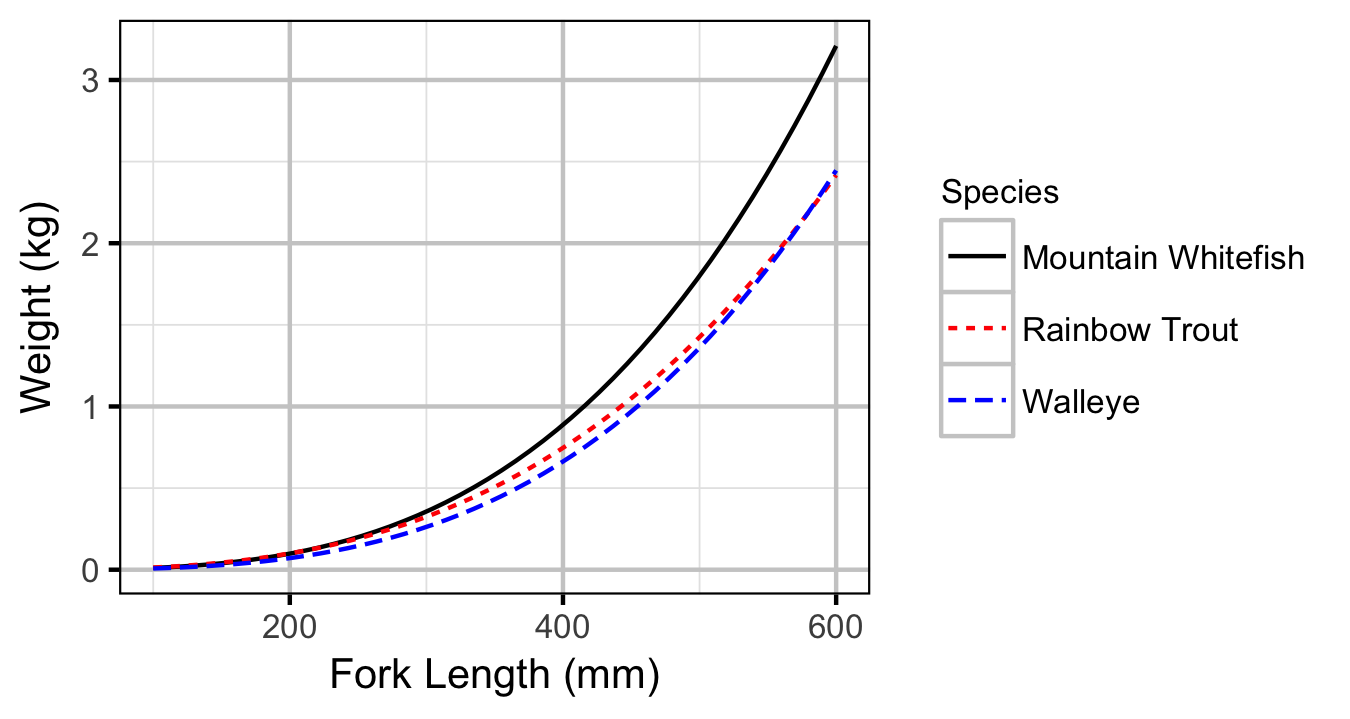Figure 1. Predicted length-mass relationship by species.

#### Subadult

##### Mountain Whitefish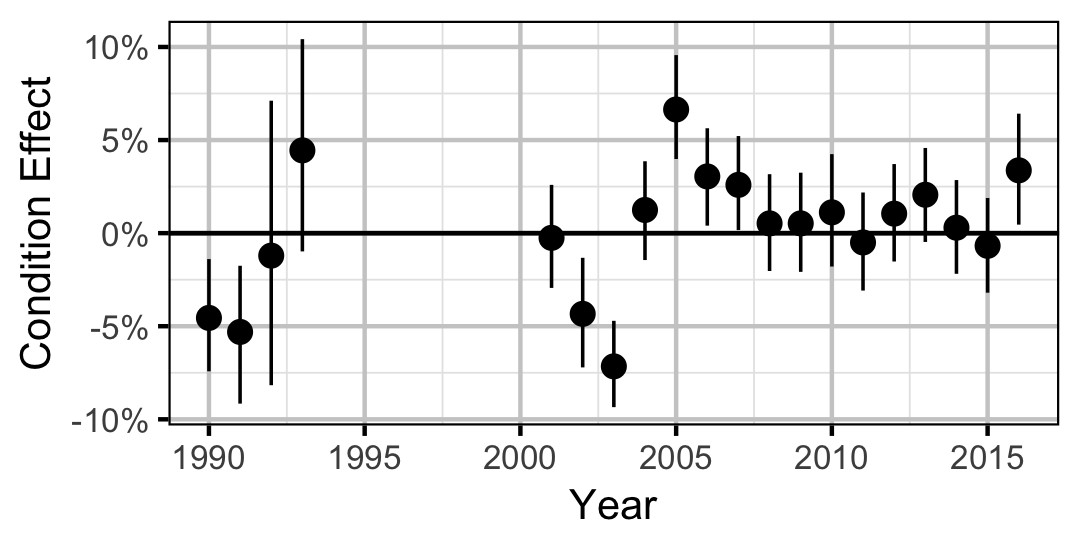Figure 2. Estimated change in condition relative to a typical year for a 200 mm Mountain Whitefish by year (with 95% CRIs).
##### Rainbow Trout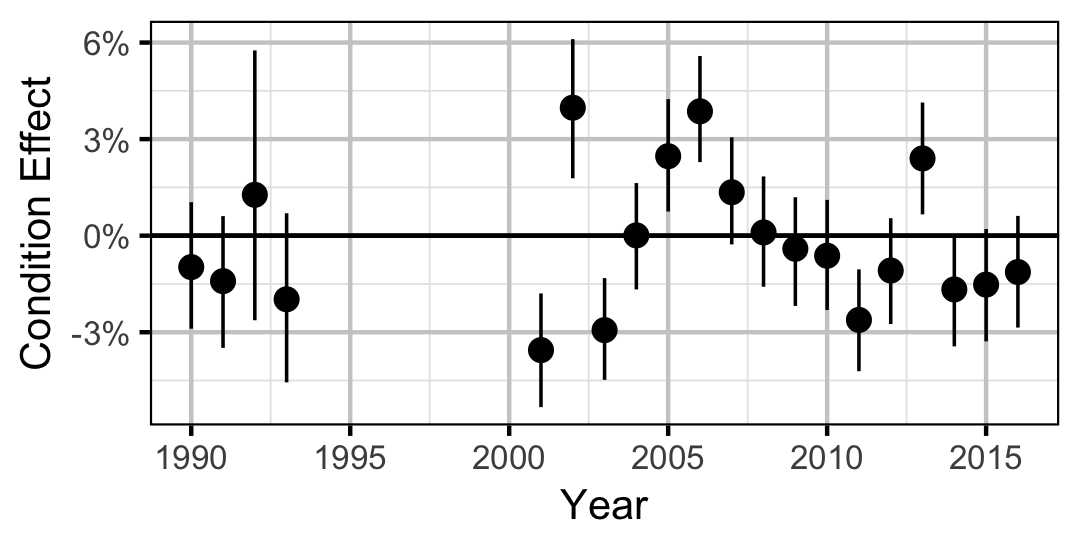Figure 3. Estimated change in condition relative to a typical year for a 250 mm Rainbow Trout by year (with 95% CRIs).

#### Adult

##### Mountain Whitefish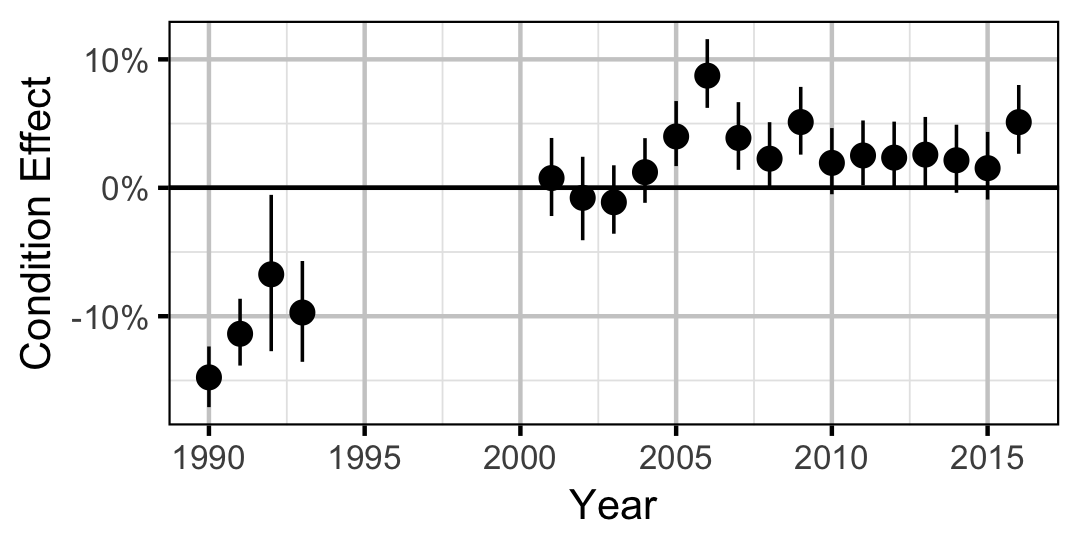Figure 4. Estimated change in condition relative to a typical year for a 350 mm Mountain Whitefish by year (with 95% CRIs).
##### Rainbow Trout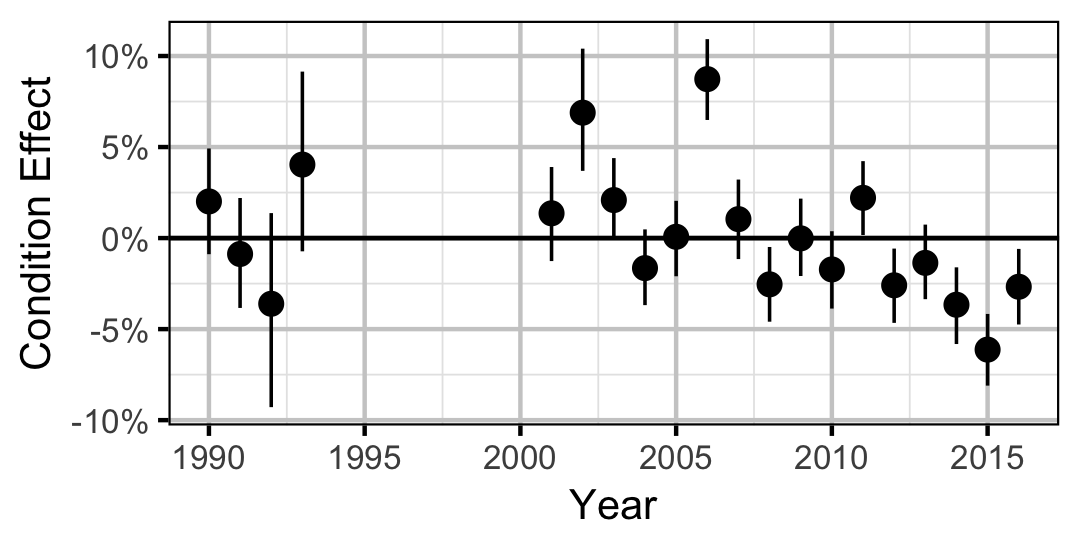Figure 5. Estimated change in condition relative to a typical year for a 500 mm Rainbow Trout by year (with 95% CRIs).
##### Walleye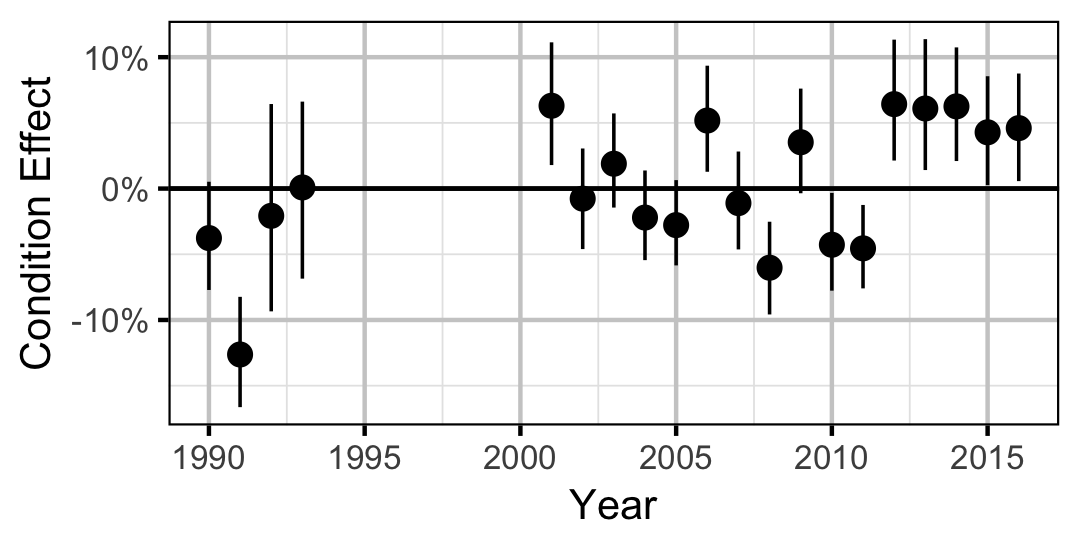Figure 6. Estimated change in condition relative to a typical year for a 600 mm Walleye by year (with 95% CRIs).

### Growth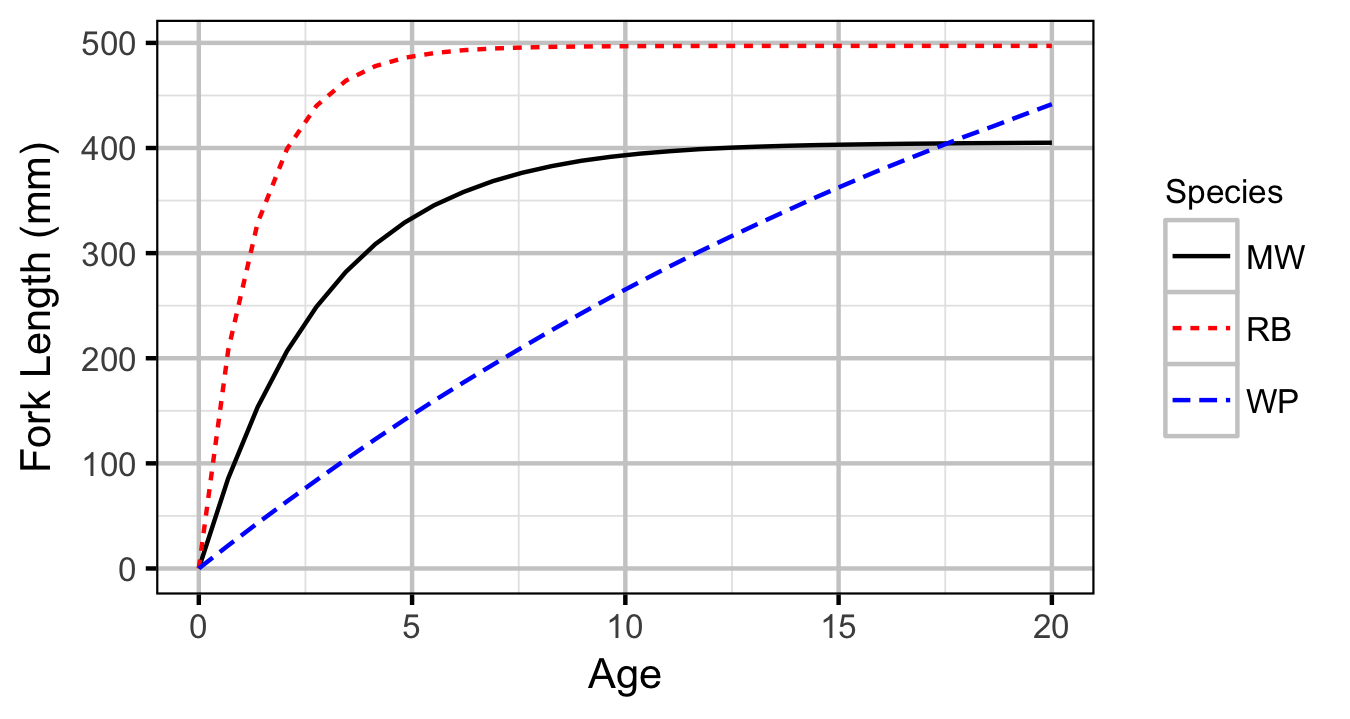Figure 7. Estimated von Bertalanffy growth curve by species.

#### Mountain Whitefish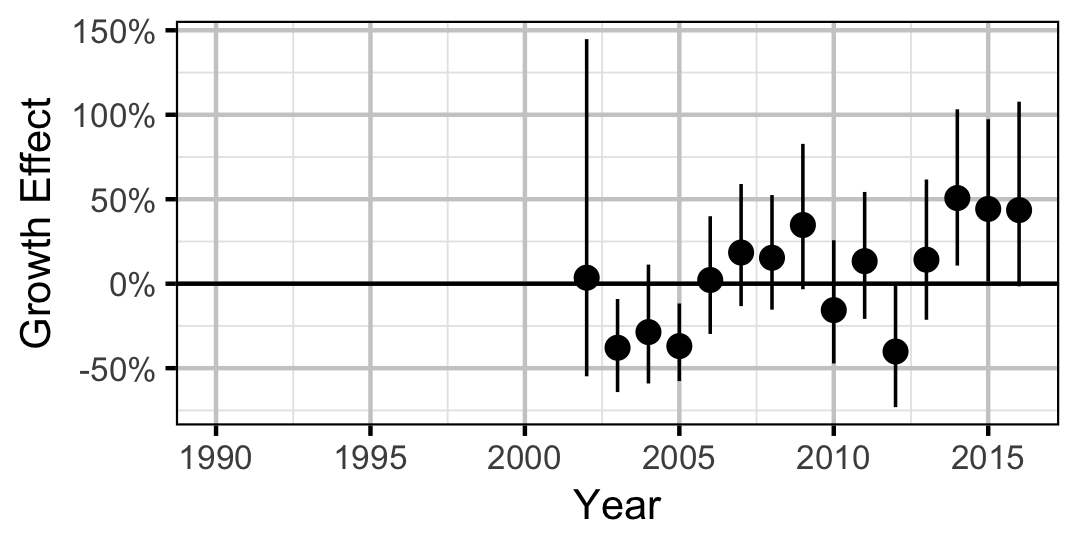Figure 8. Estimated change in von Bertalanffy growth coefficient (k) relative to a typical year by year (with 95% CIs).

#### Rainbow Trout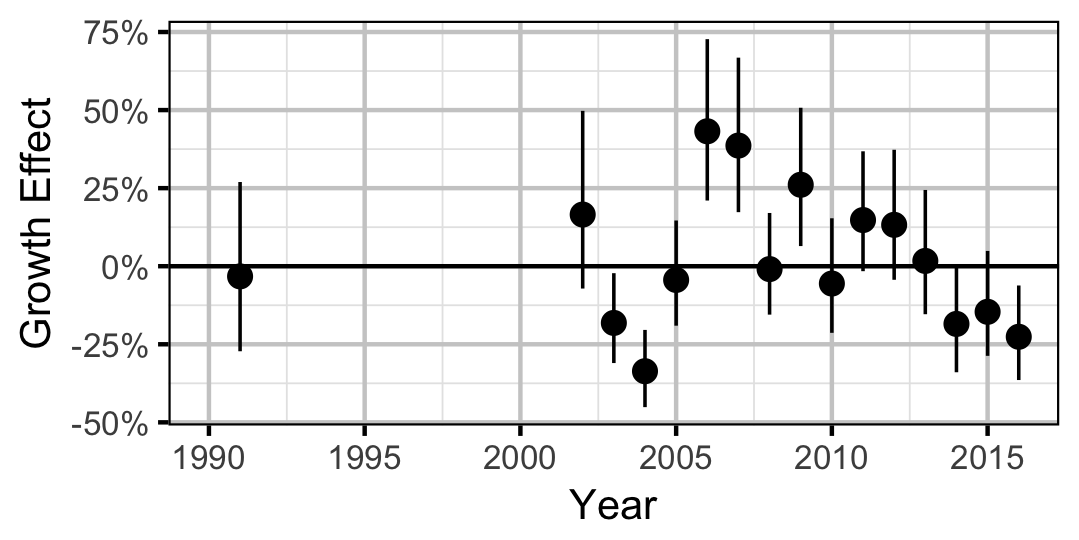Figure 9. Estimated change in von Bertalanffy growth coefficient (k) relative to a typical year by year (with 95% CIs).

#### Walleye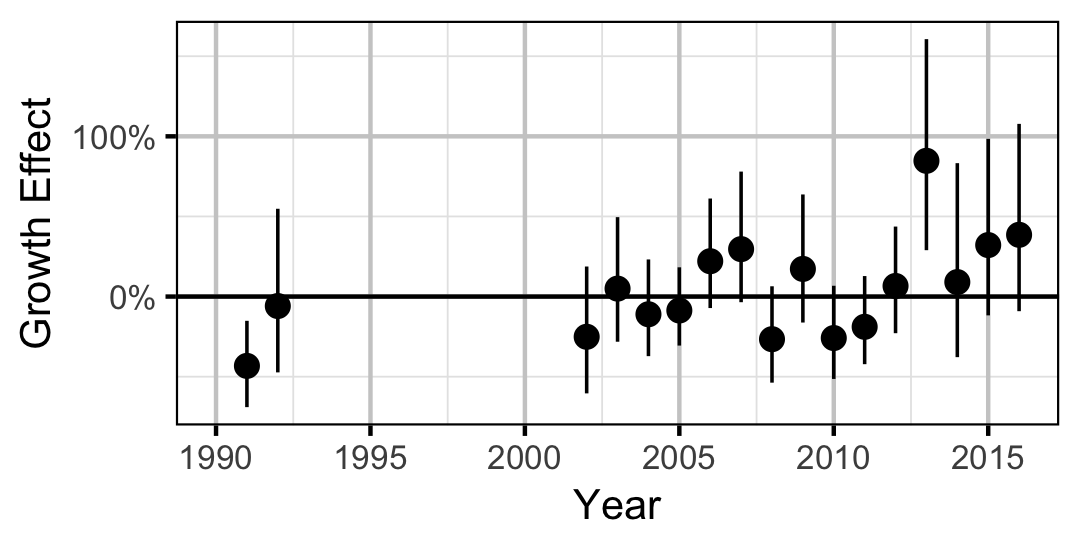Figure 10. Estimated change in von Bertalanffy growth coefficient (k) relative to a typical year by year (with 95% CIs).

### Length-At-Age

#### Mountain Whitefish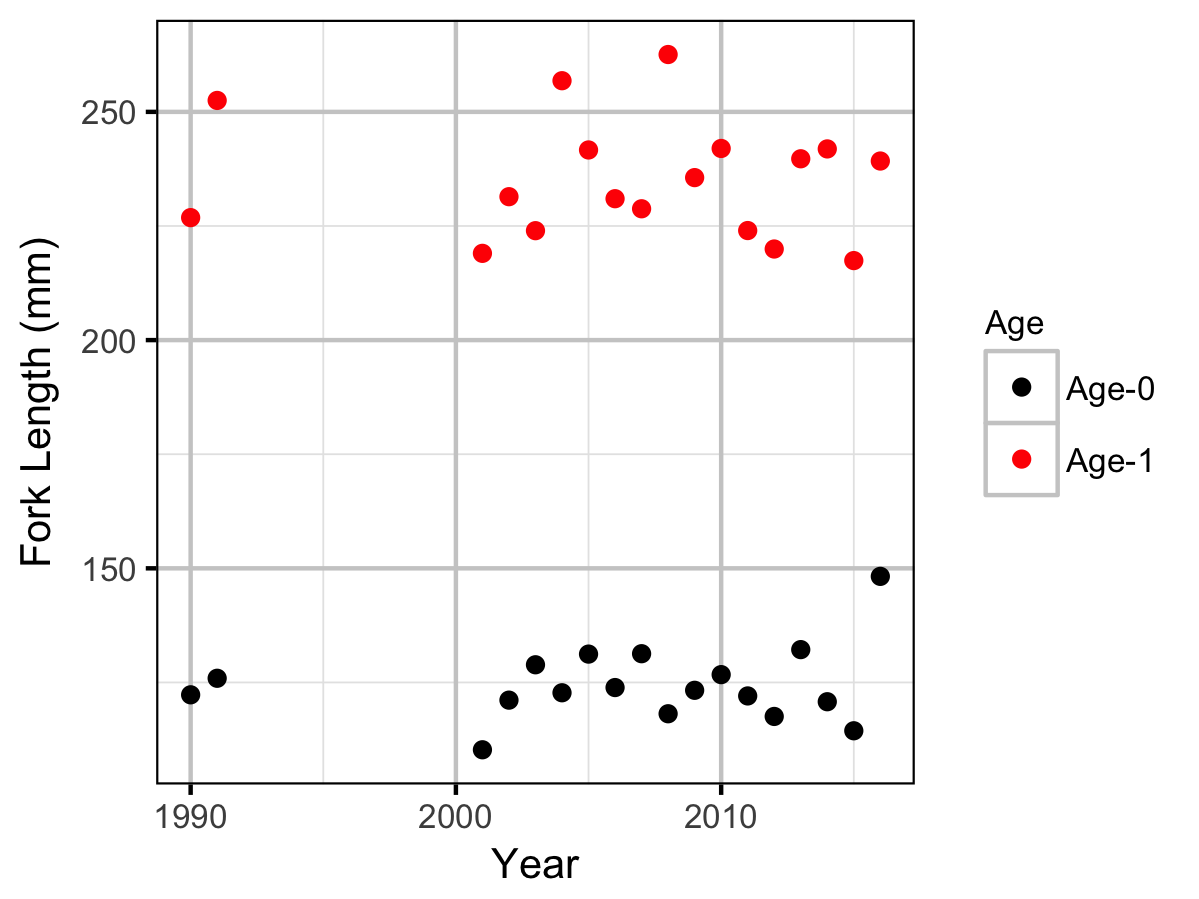Figure 11. Mean fork length (mm) of different age classes for Mountain Whitefishby year.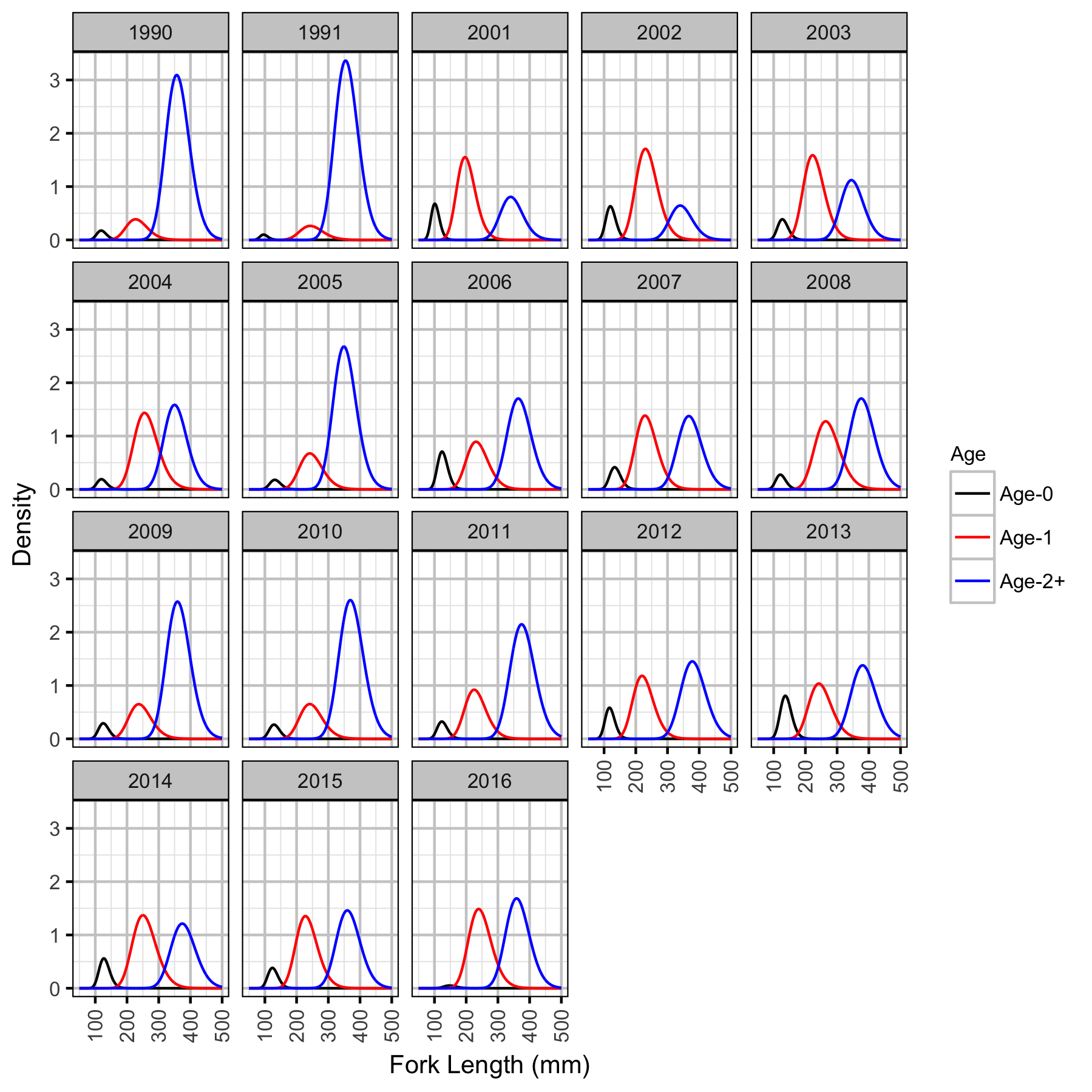Figure 12. Predicted length-density plot for Mountain Whitefish by life-stage and year.

#### Rainbow Trout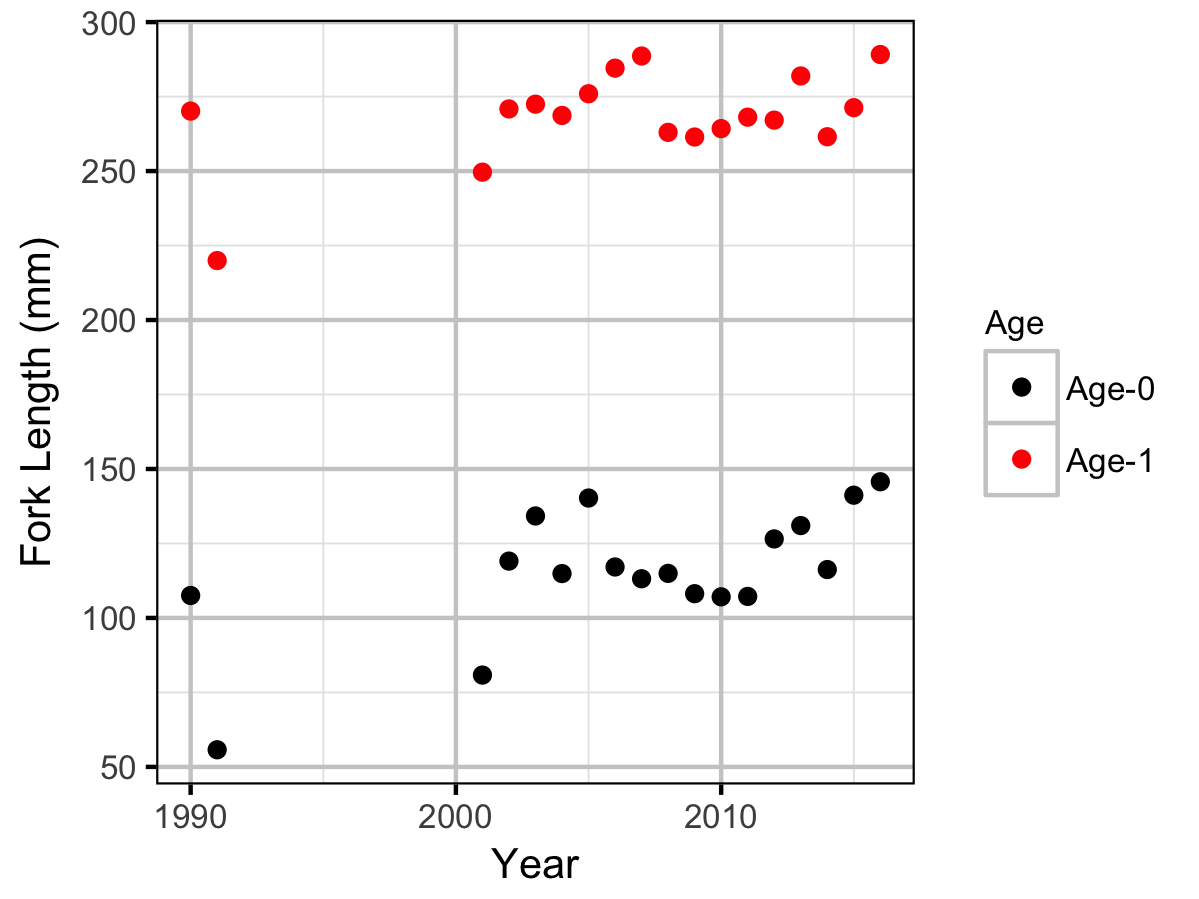Figure 13. Mean fork length (mm) of different age classes for Rainbow Troutby year.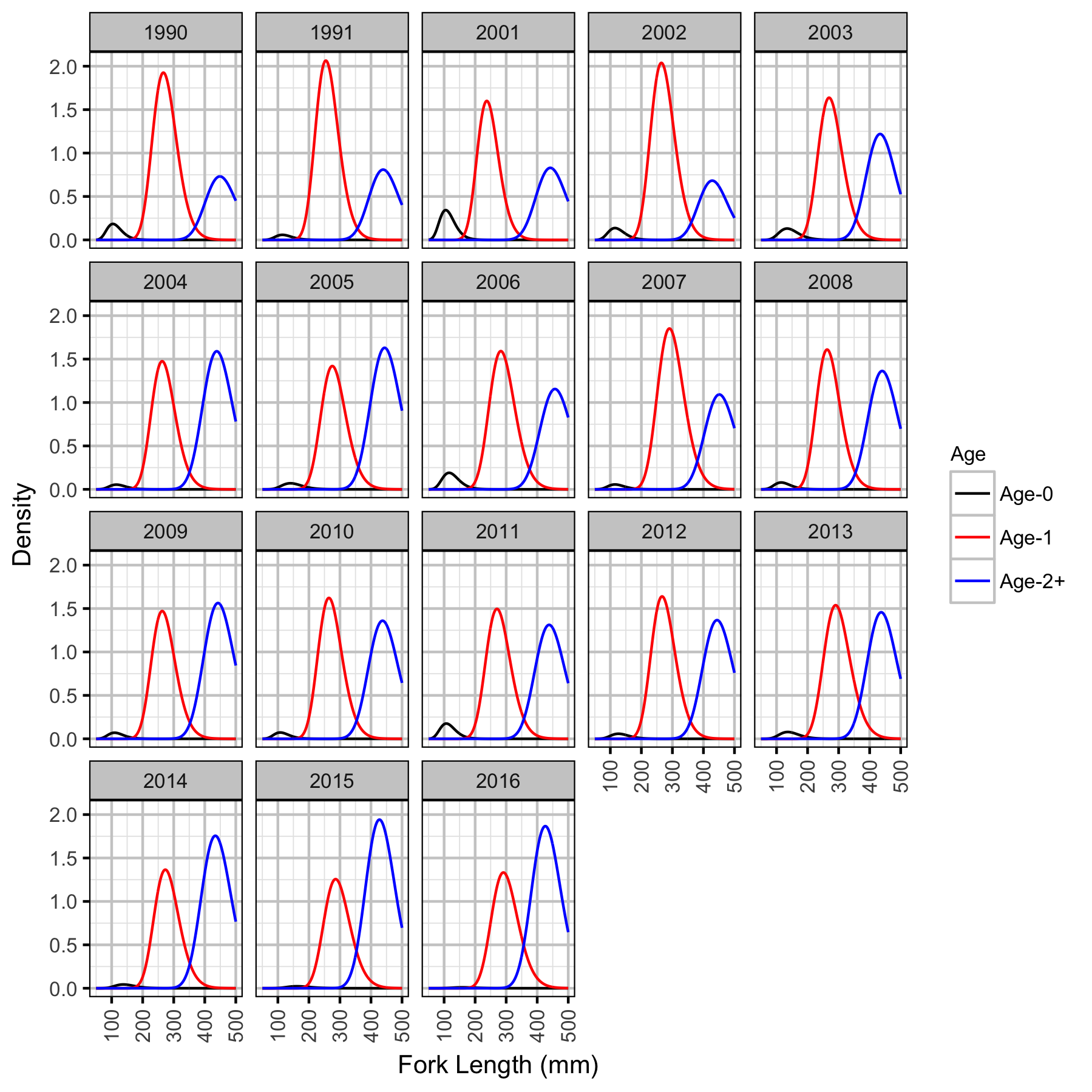Figure 14. Predicted length-density plot for Rainbow Trout by life-stage and year.

### Observer Length Correction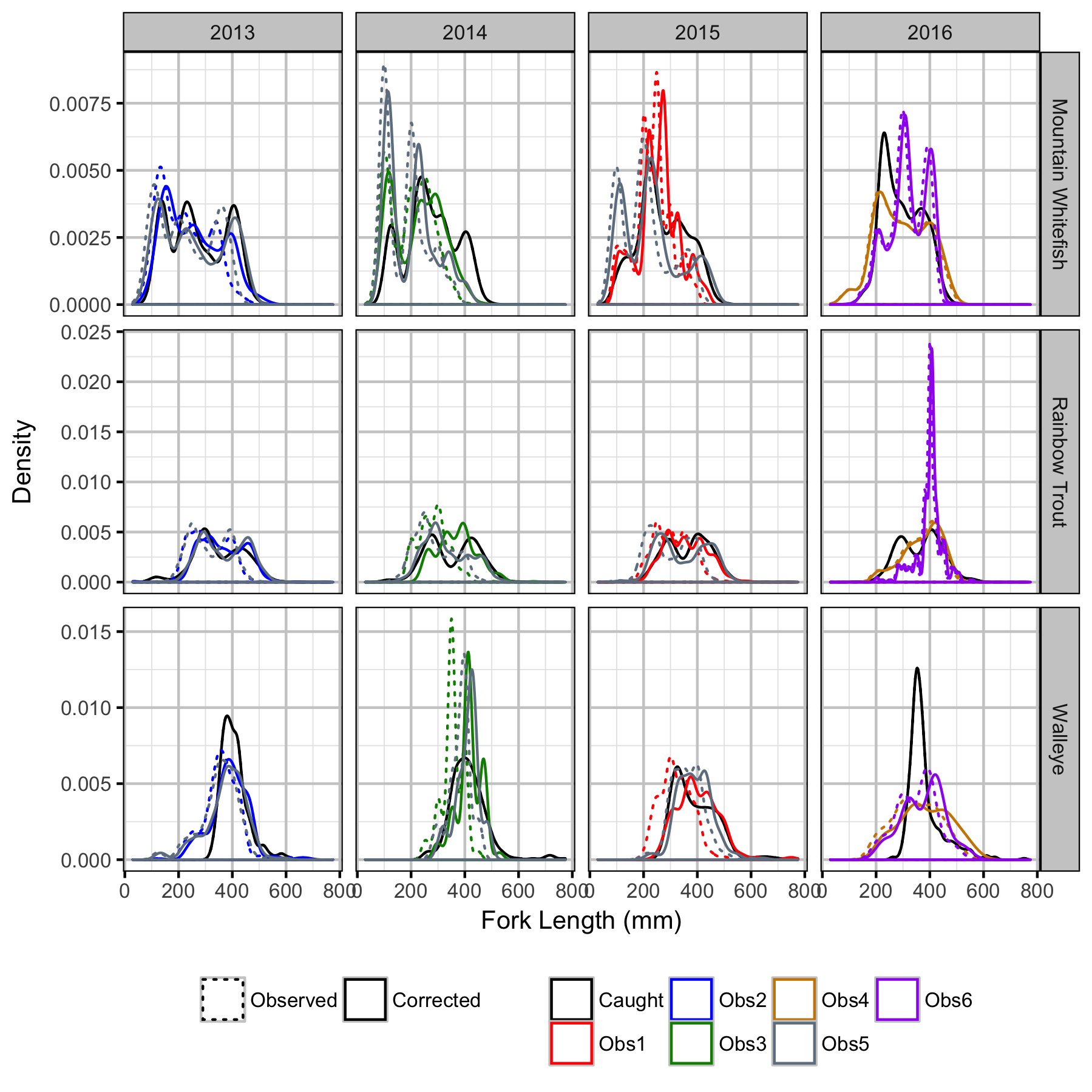Figure 15. Observed and corrected length density plots for measured versus counted fish by observer and species.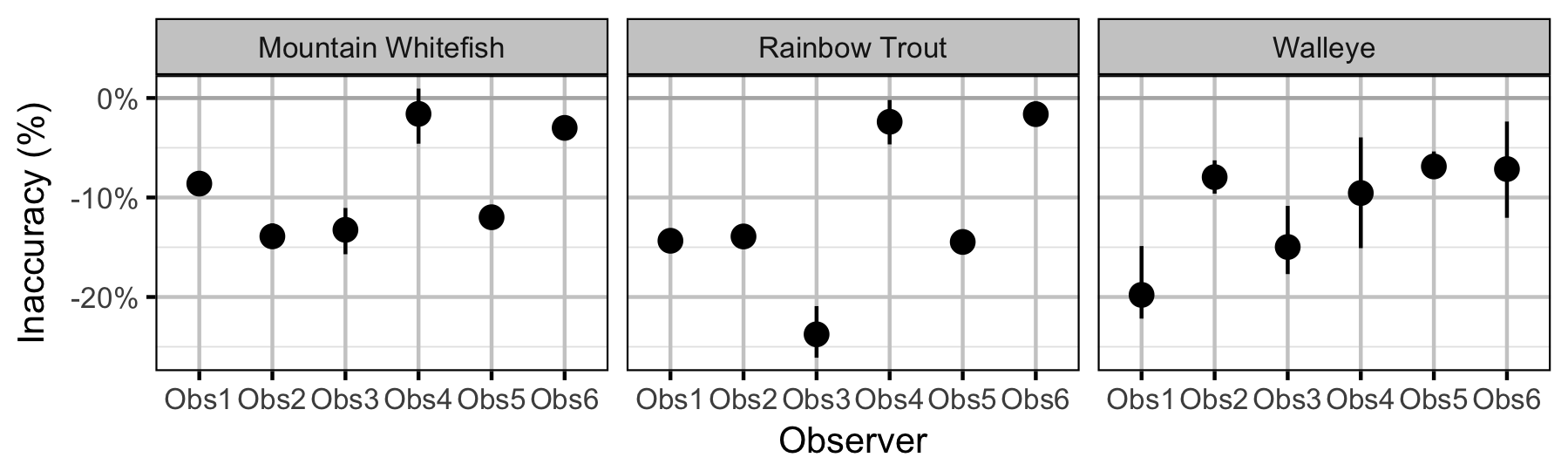Figure 16. Predicted length inaccuracy relative to measured by observer and species (with 95% CRIs).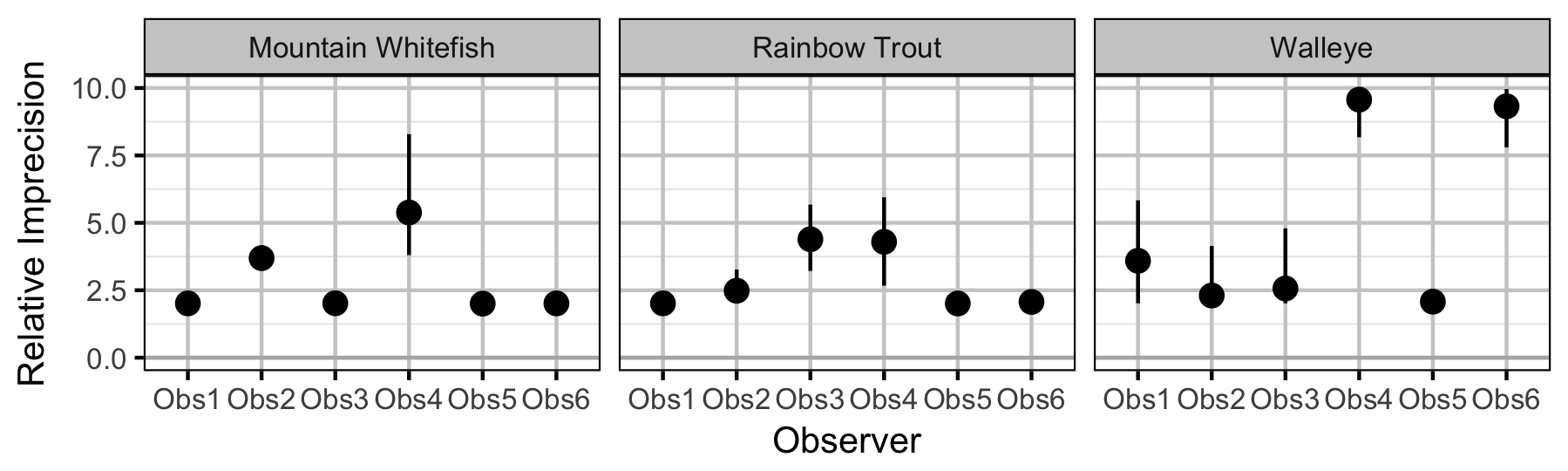Figure 17. Predicted imprecision relative to measured by observer and species (with 95% CRIs).

### Survival

#### Adult

##### Mountain Whitefish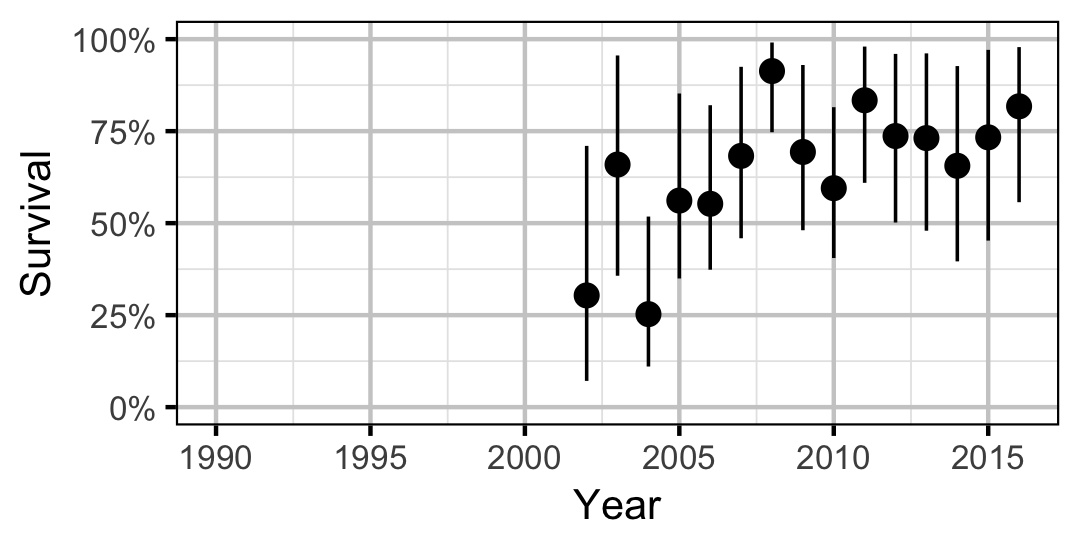Figure 18. Predicted annual survival for an adult Mountain Whitefish.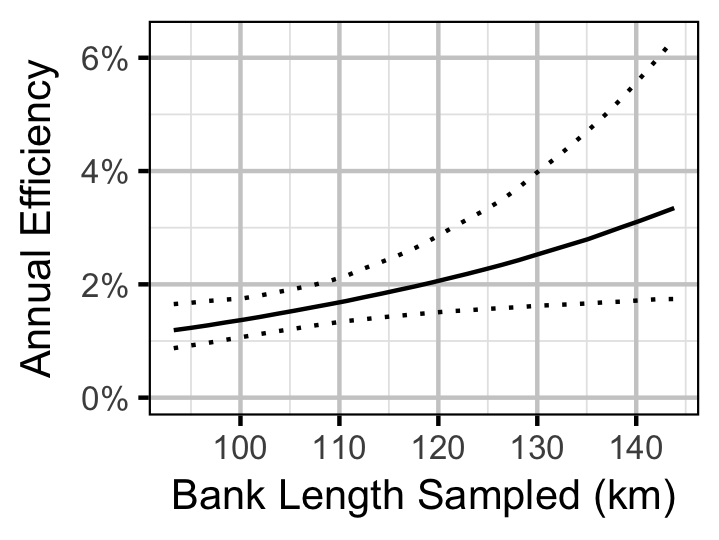Figure 19. Predicted annual efficiency for an adult Mountain Whitefish.
##### Rainbow Trout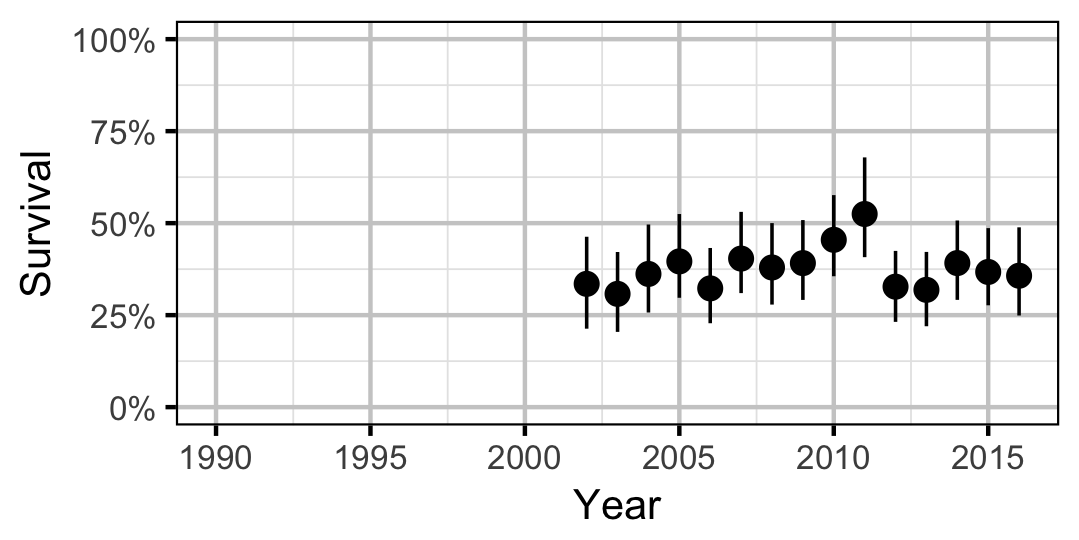Figure 20. Predicted annual survival for an adult Rainbow Trout.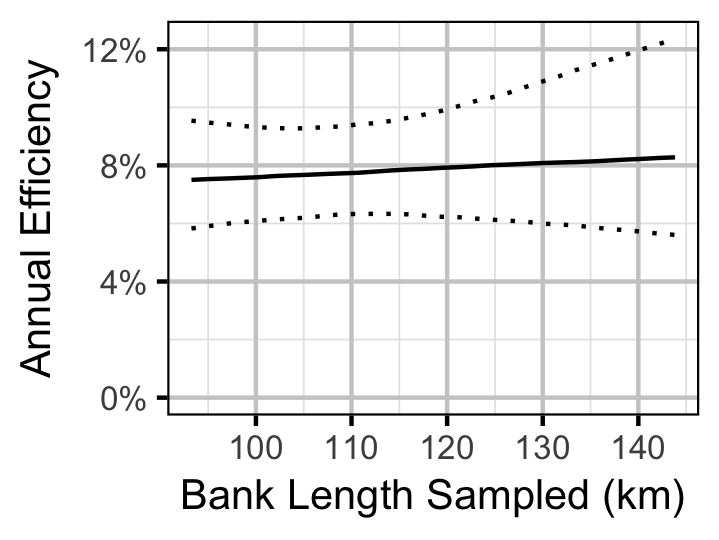Figure 21. Predicted annual efficiency for an adult Rainbow Trout.
##### Walleye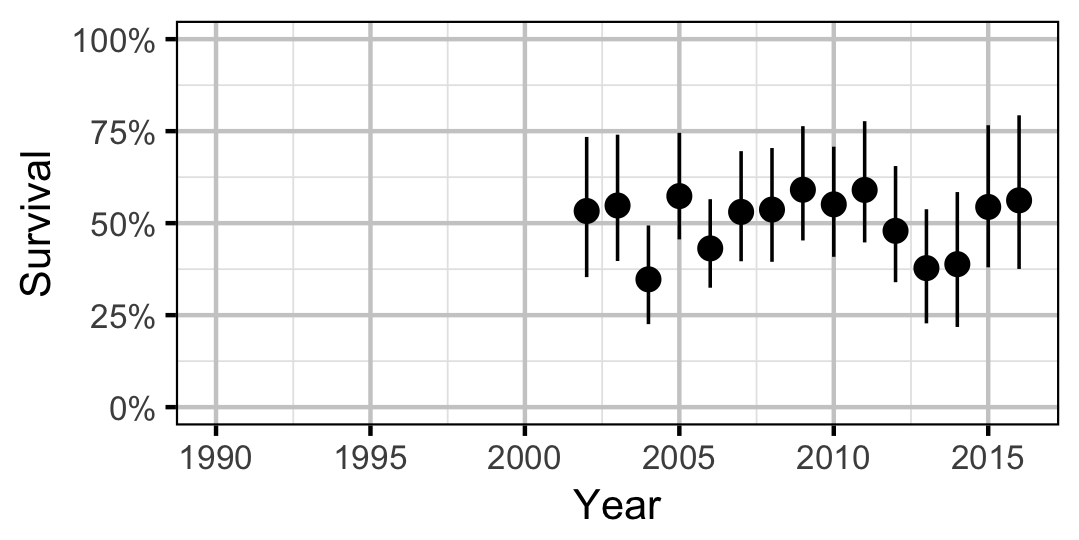Figure 22. Predicted annual survival for an adult Walleye.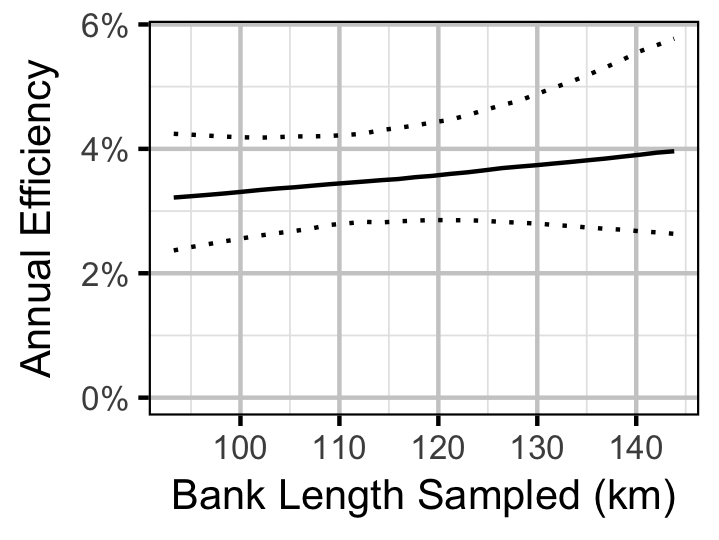Figure 23. Predicted annual efficiency for an adult Walleye.

### Site Fidelity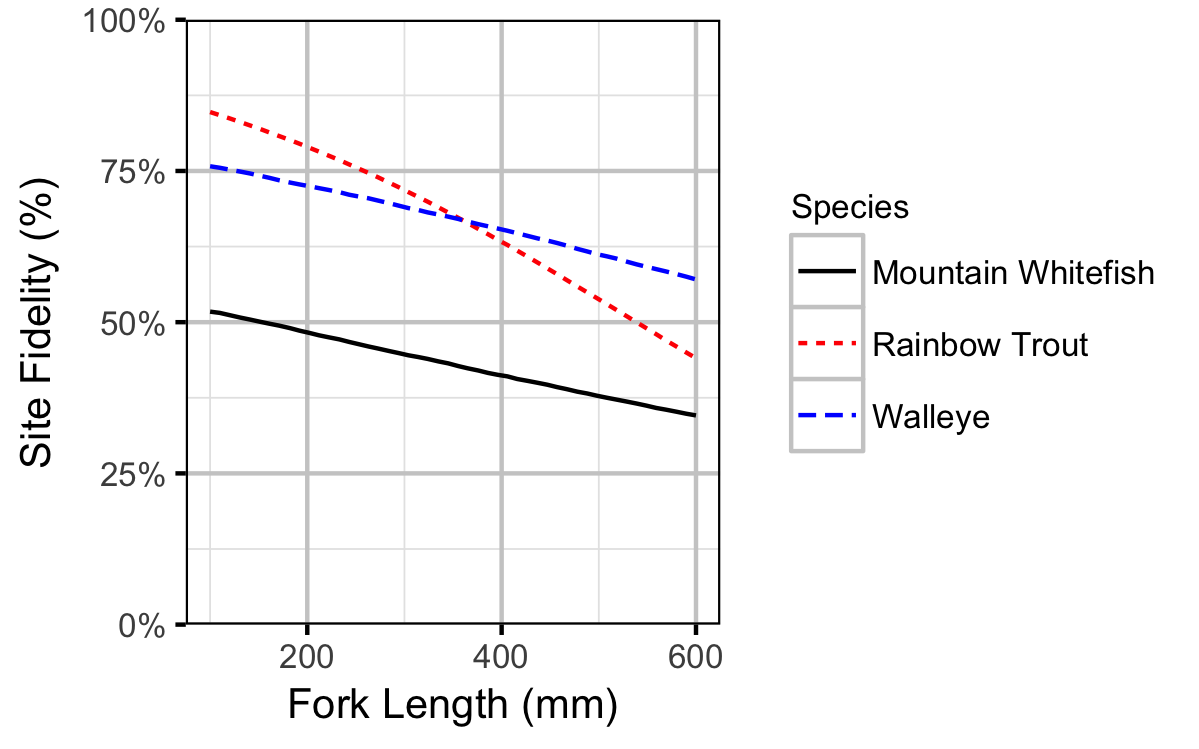Figure 24. Probability of recapture at the same site versus a different site by fish length (with 95% CRIs).

### Capture Efficiency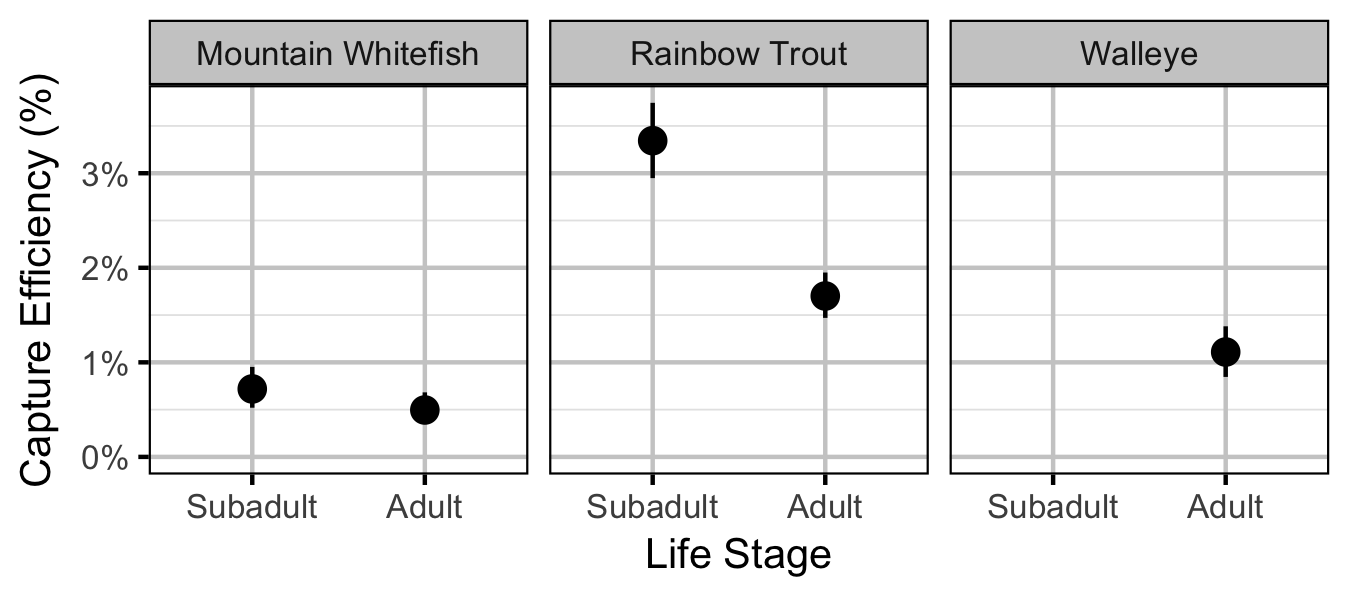Figure 25. Predicted capture efficiency by species and life stage (with 95% CRIs).

#### Mountain Whitefish

##### Subadult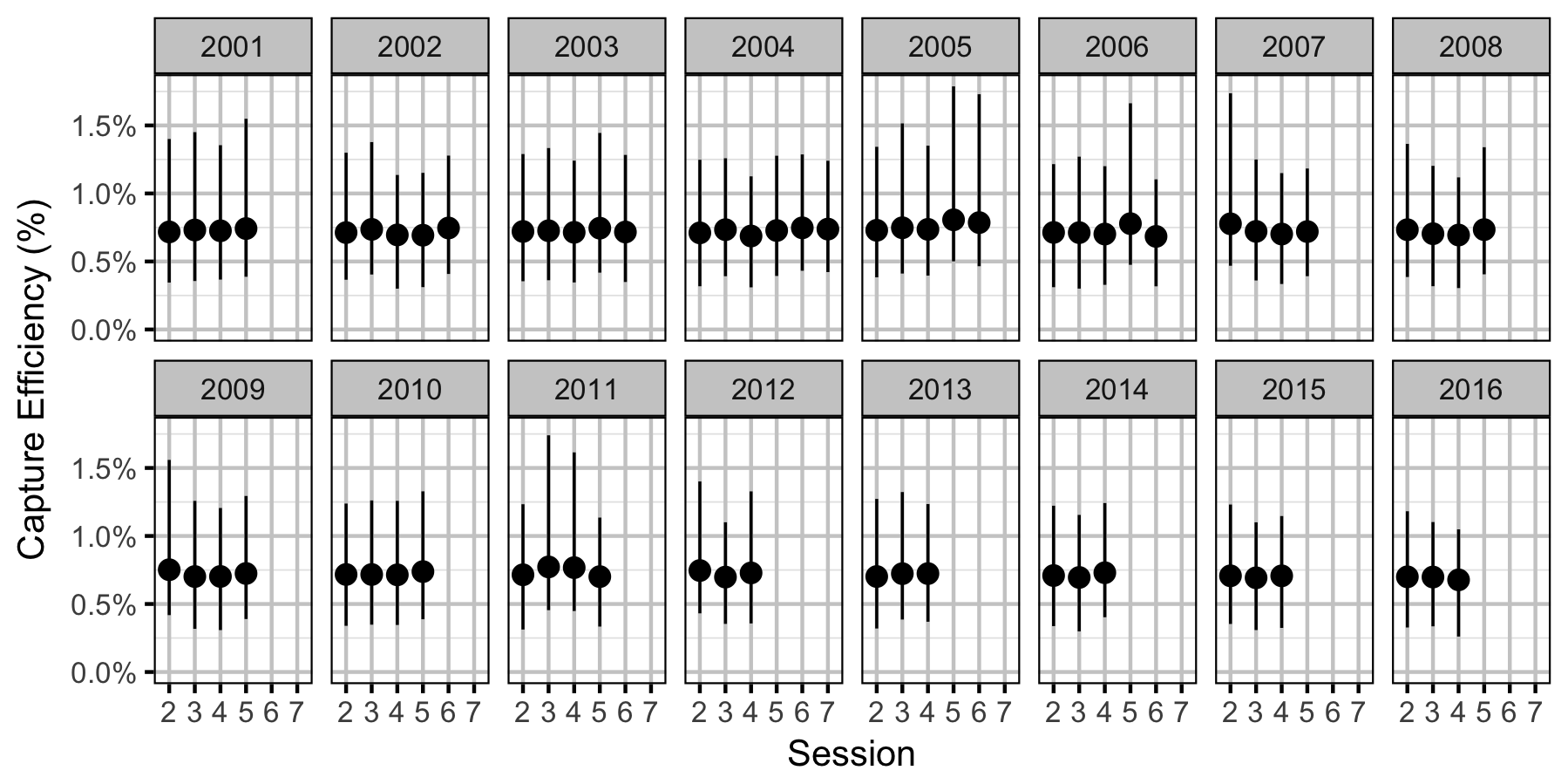Figure 26. Predicted capture efficiency for a subadult Mountain Whitefish by session and year (with 95% CRIs).
##### Adult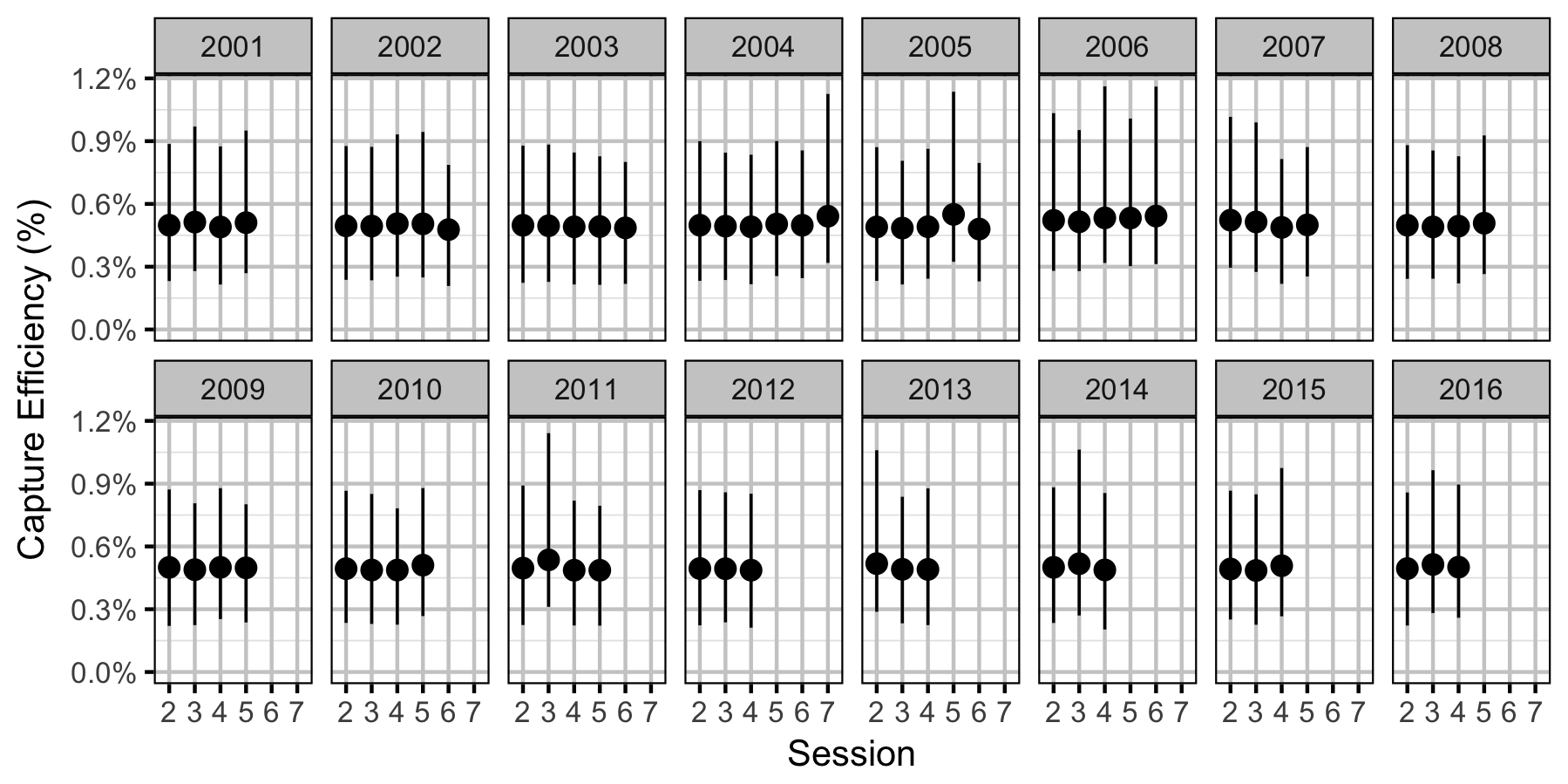Figure 27. Predicted capture efficiency for an adult Mountain Whitefish by session and year (with 95% CRIs).

#### Rainbow Trout

##### Subadult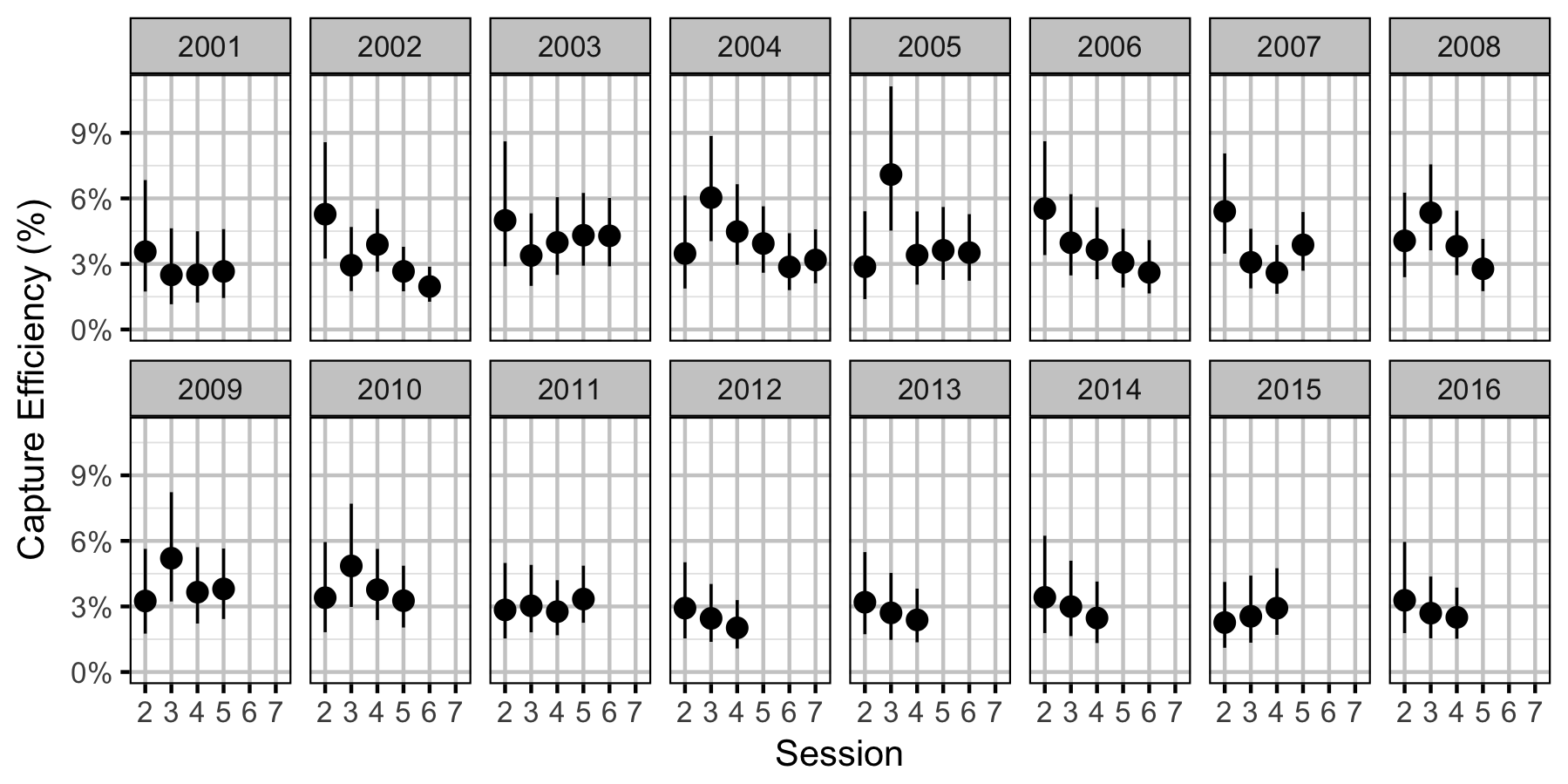Figure 28. Predicted capture efficiency for a subadult Rainbow Trout by session and year (with 95% CRIs).
##### Adult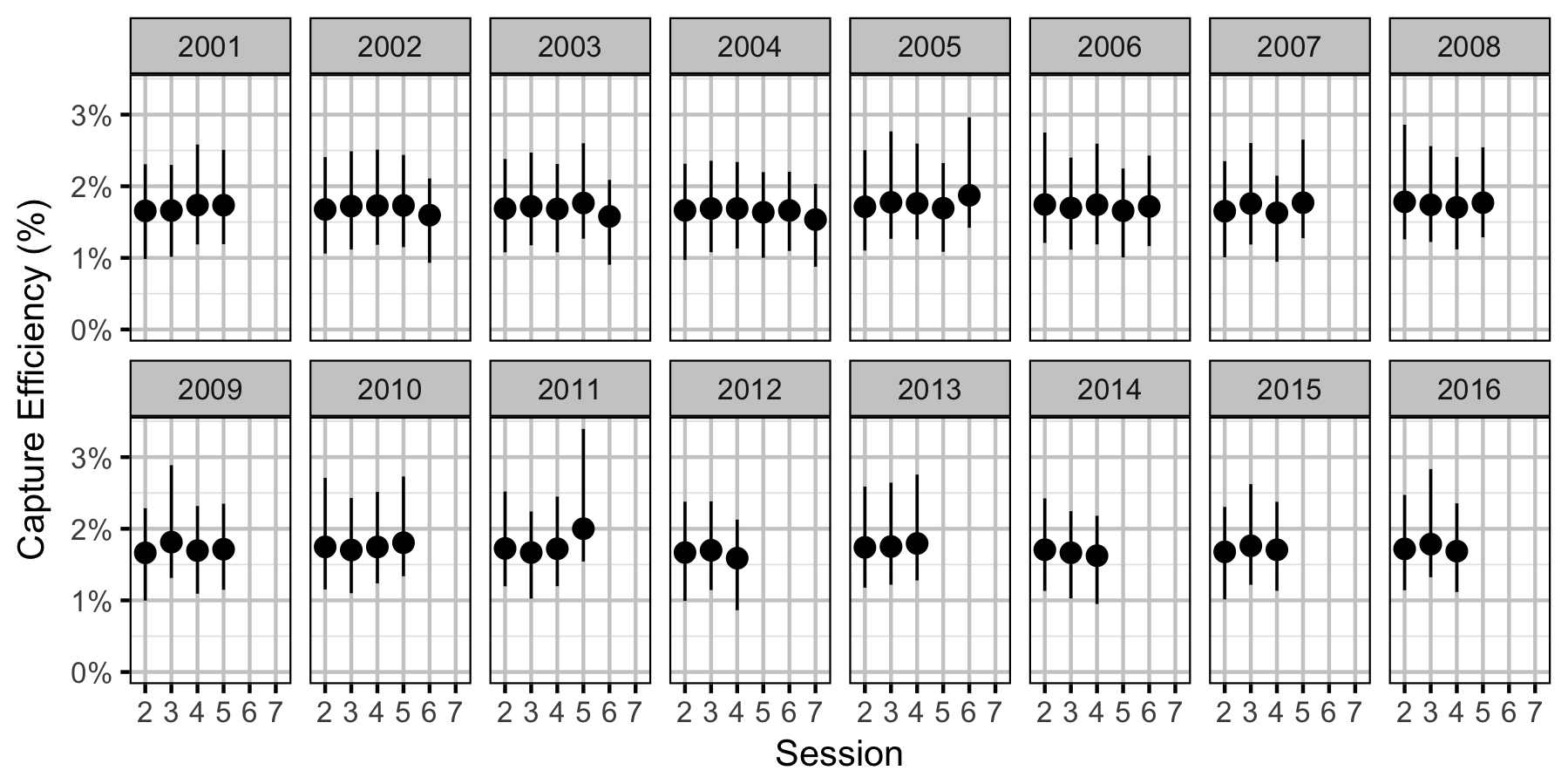Figure 29. Predicted capture efficiency for an adult Rainbow Trout by session and year (with 95% CRIs).

#### Walleye

##### Adult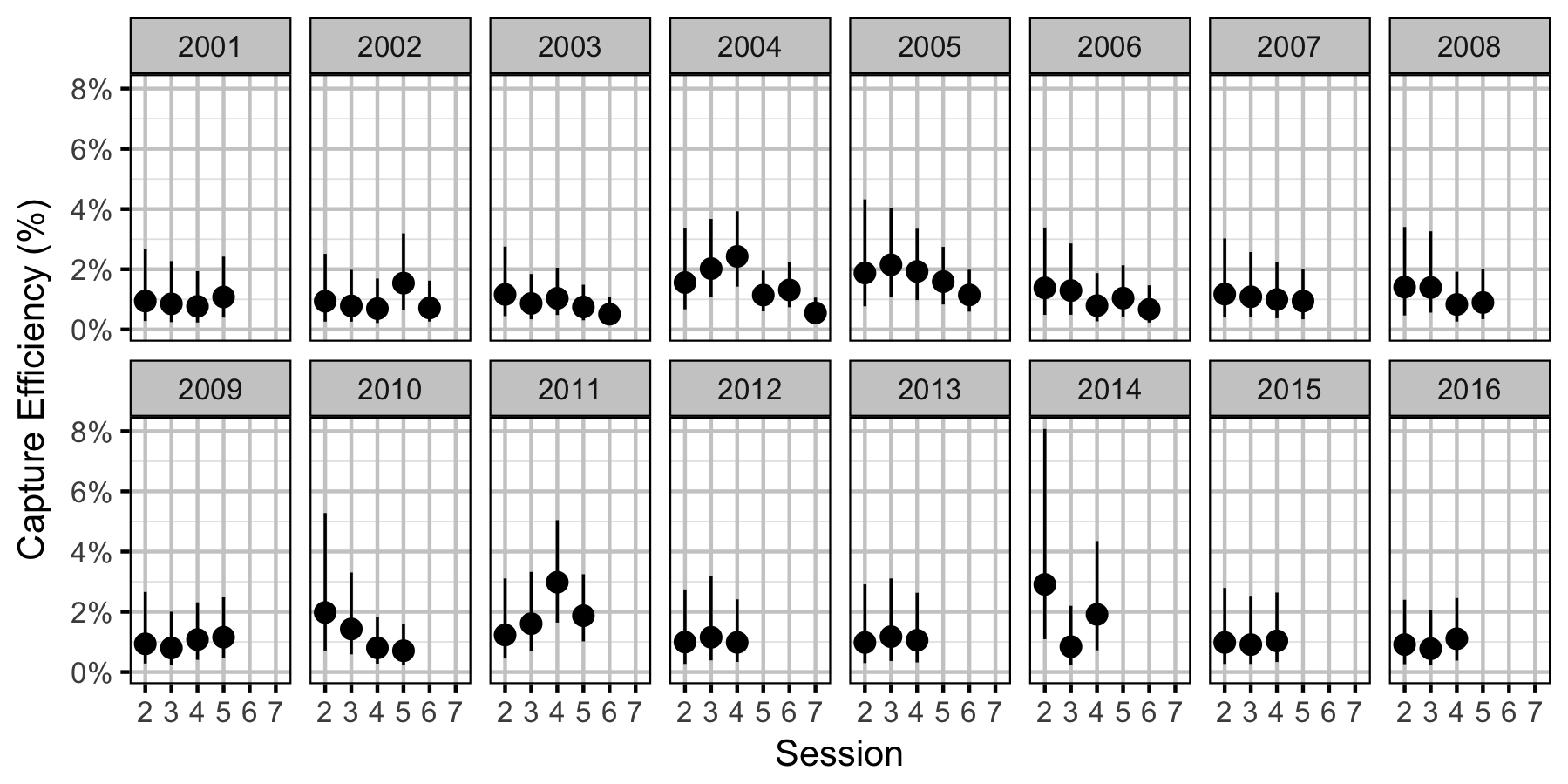Figure 30. Predicted capture efficiency for an adult Walleye by session and year (with 95% CRIs).

### Abundance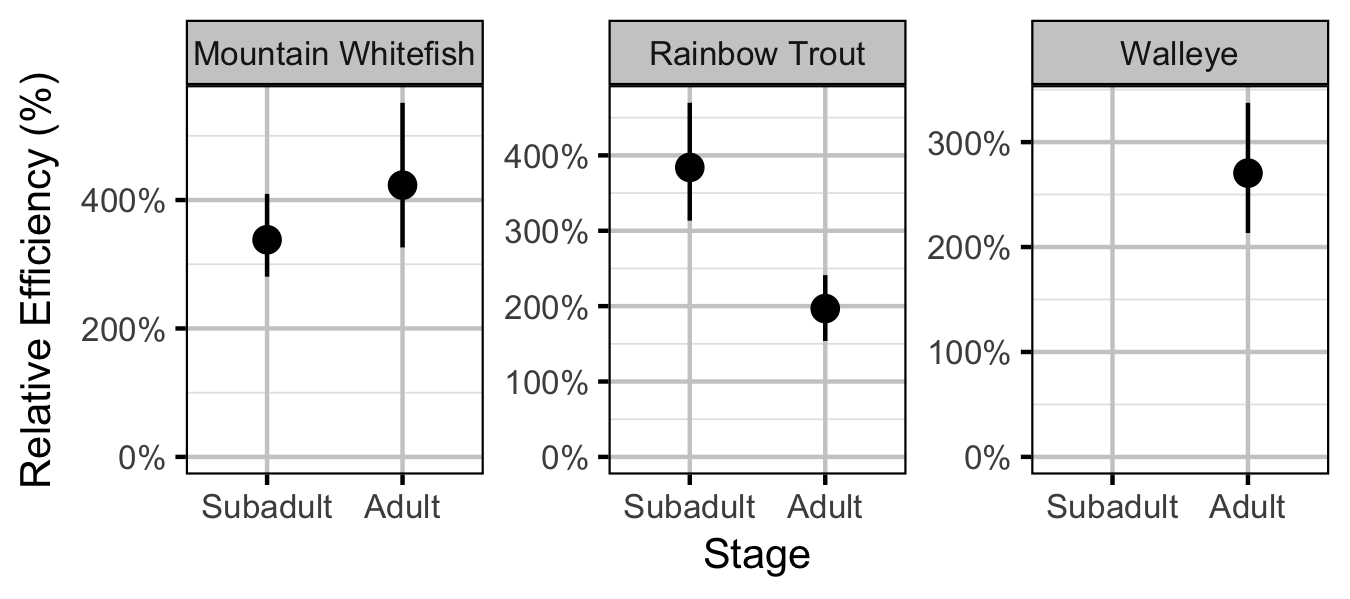Figure 31. Effect of counting (versus capture) on encounter efficiency by species and stage (with 95% CIs).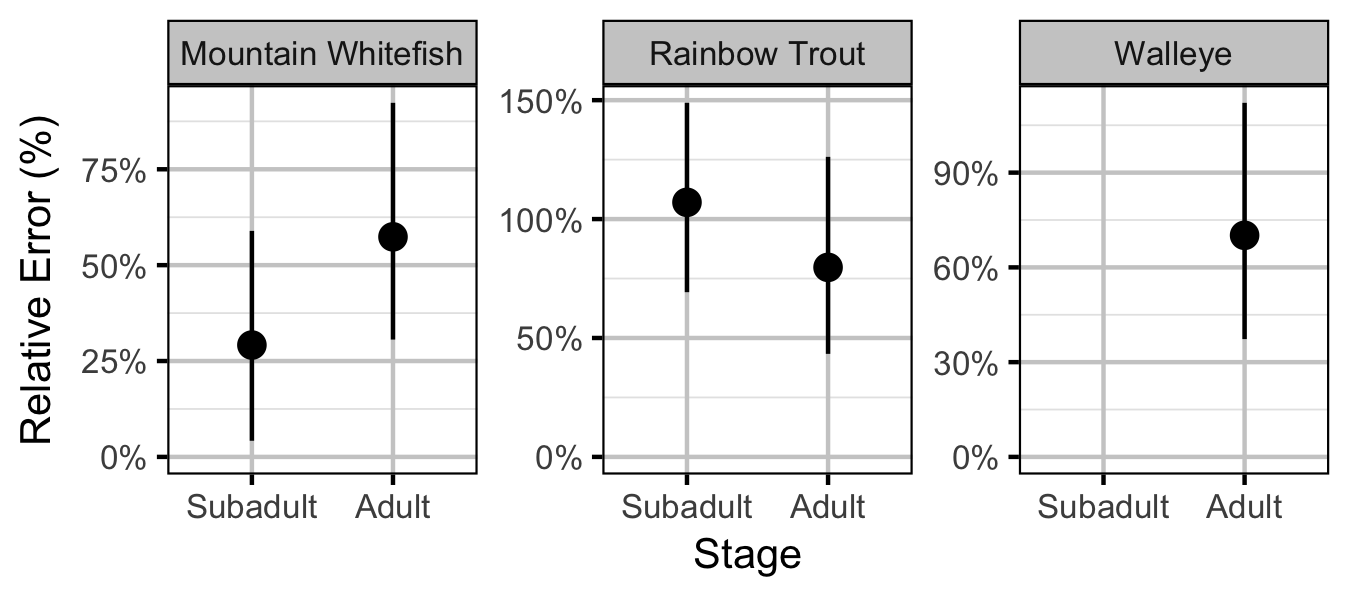Figure 32. Effect of counting (versus capture) on overdispersion by species and stage (with 95% CIs).

#### Mountain Whitefish

##### Subadult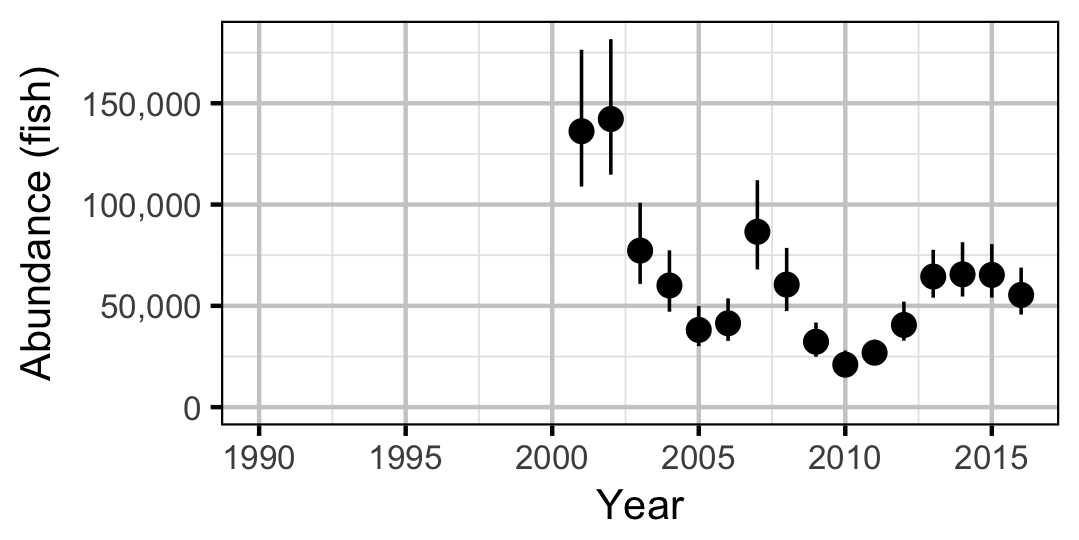Figure 33. Estimated abundance of subadult Mountain Whitefish by year (with 95% CIs).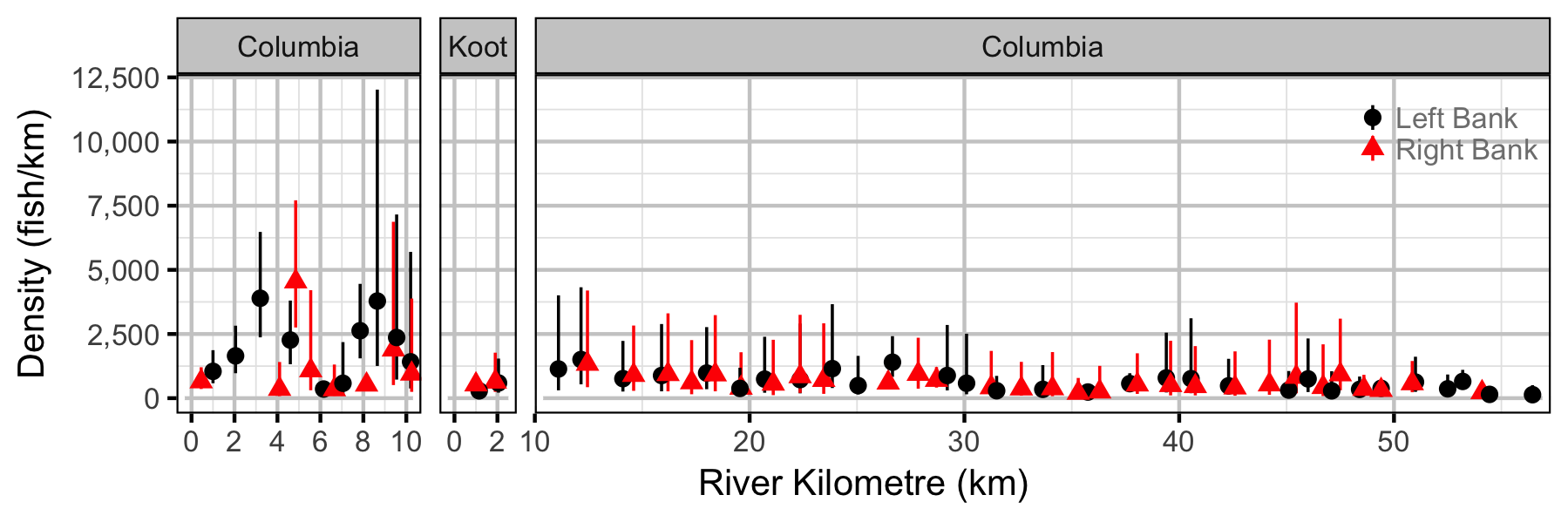Figure 34. Estimated lineal river count density of subadult Mountain Whitefish by site in (with 95% CIs).
##### Adult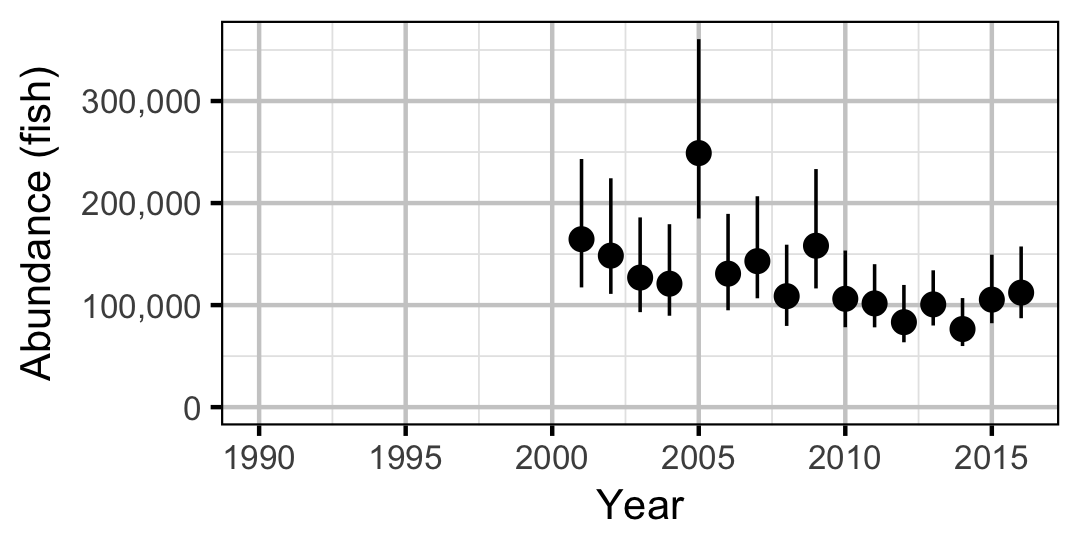Figure 35. Estimated abundance of adult Mountain Whitefish by year (with 95% CIs).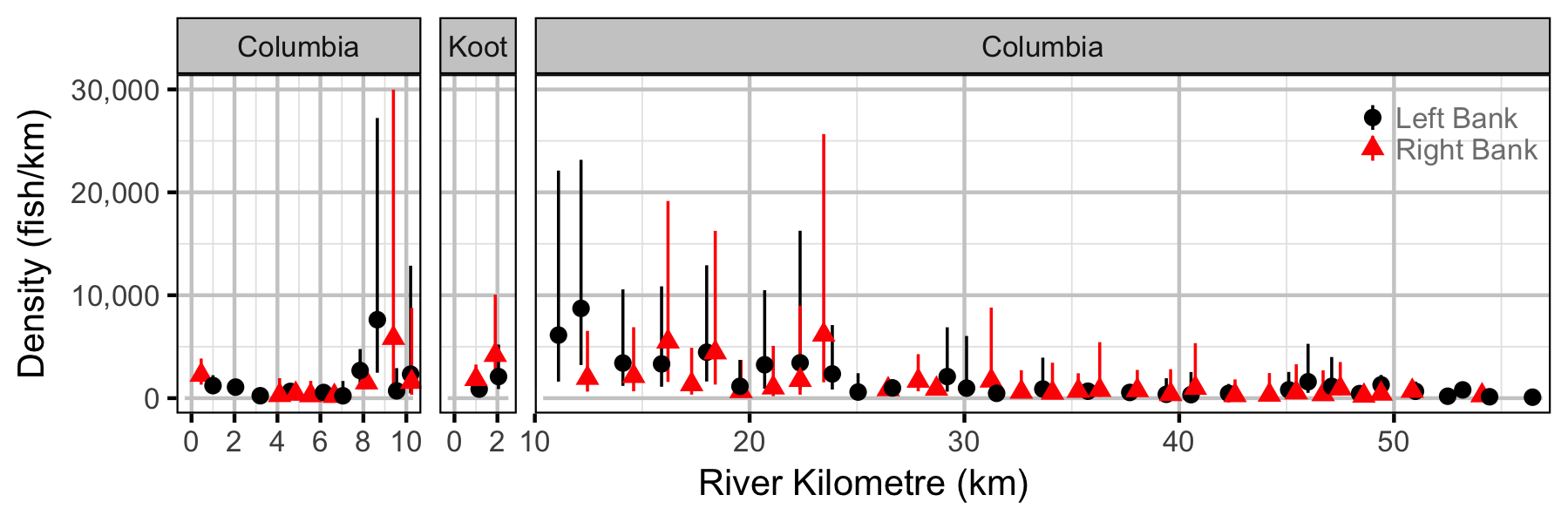Figure 36. Estimated lineal river count density of adult Mountain Whitefish by site in (with 95% CIs).

#### Rainbow Trout

##### Subadult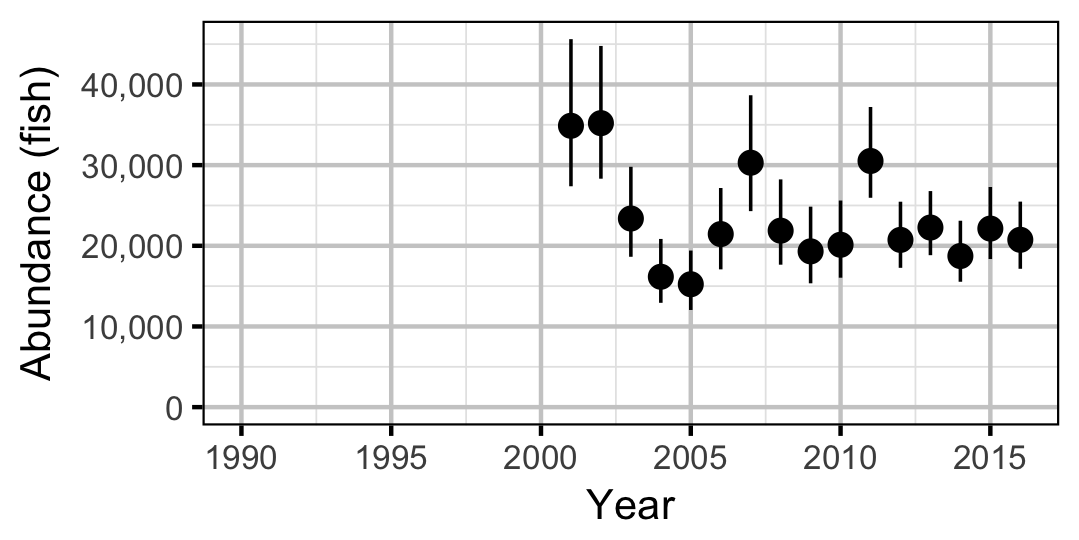Figure 37. Estimated abundance of subadult Rainbow Trout by year (with 95% CIs).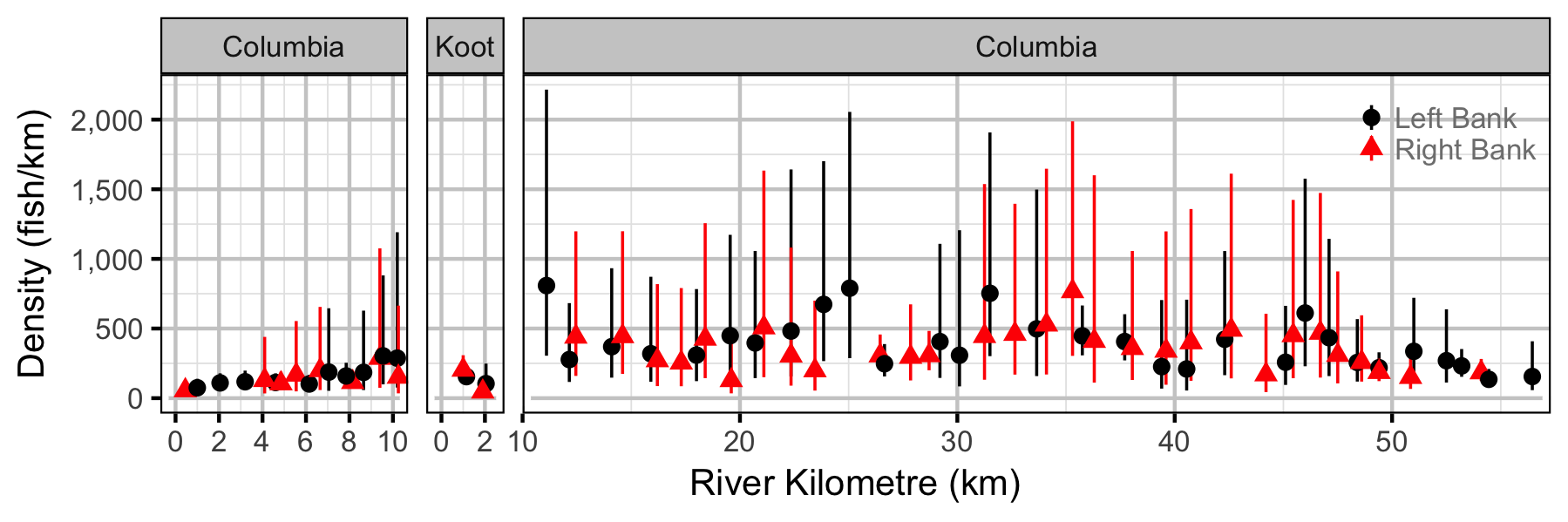Figure 38. Estimated lineal river count density of subadult Rainbow Trout by site in (with 95% CIs).
##### Adult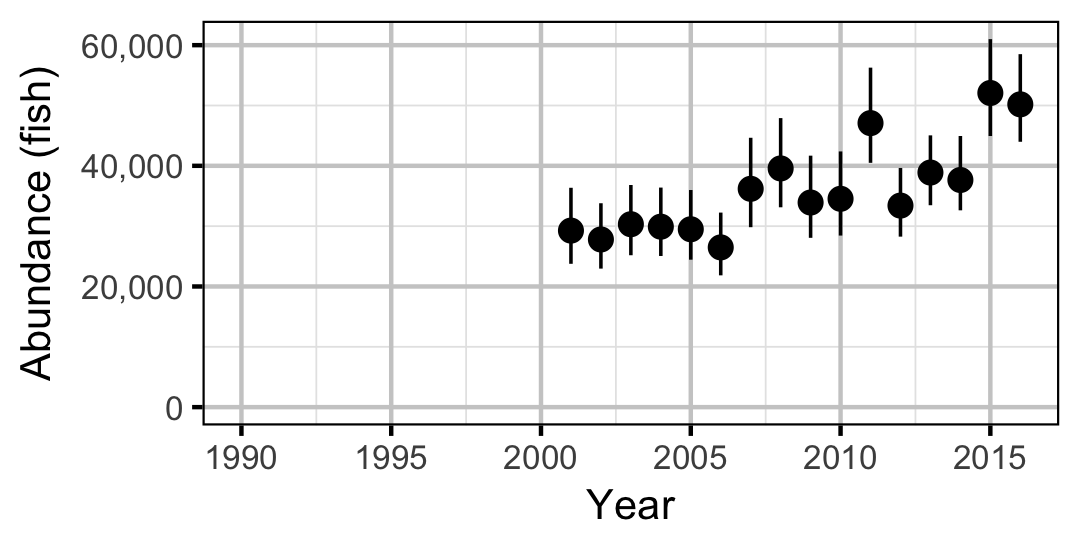Figure 39. Estimated abundance of adult Rainbow Trout by year (with 95% CIs).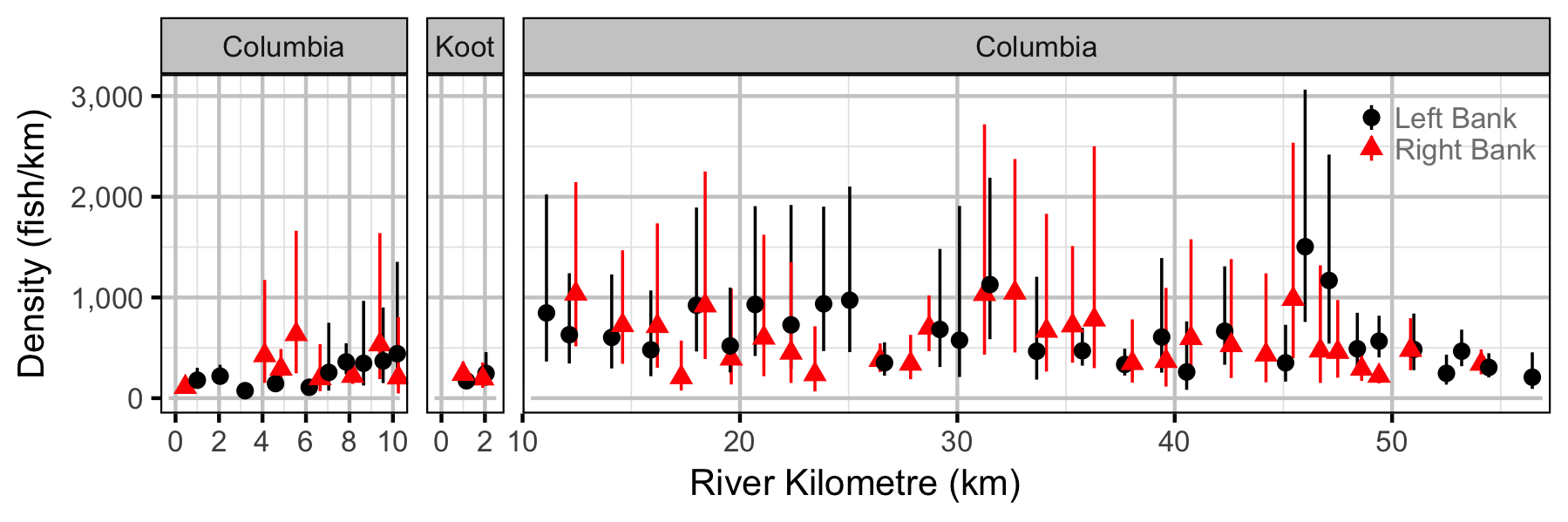Figure 40. Estimated lineal river count density of adult Rainbow Trout by site in (with 95% CIs).

#### Walleye

##### Adult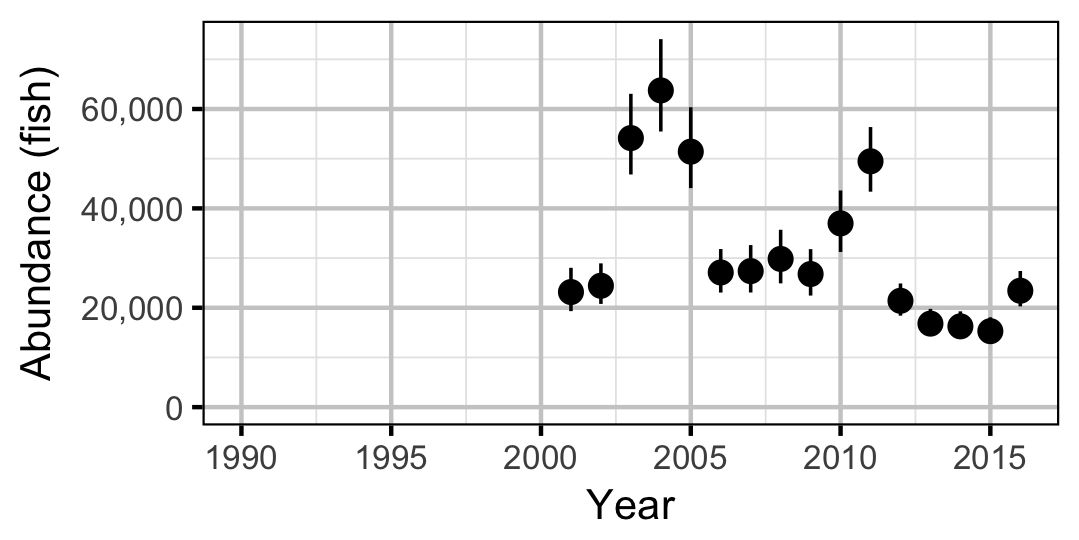Figure 41. Estimated abundance of adult Walleye by year (with 95% CIs).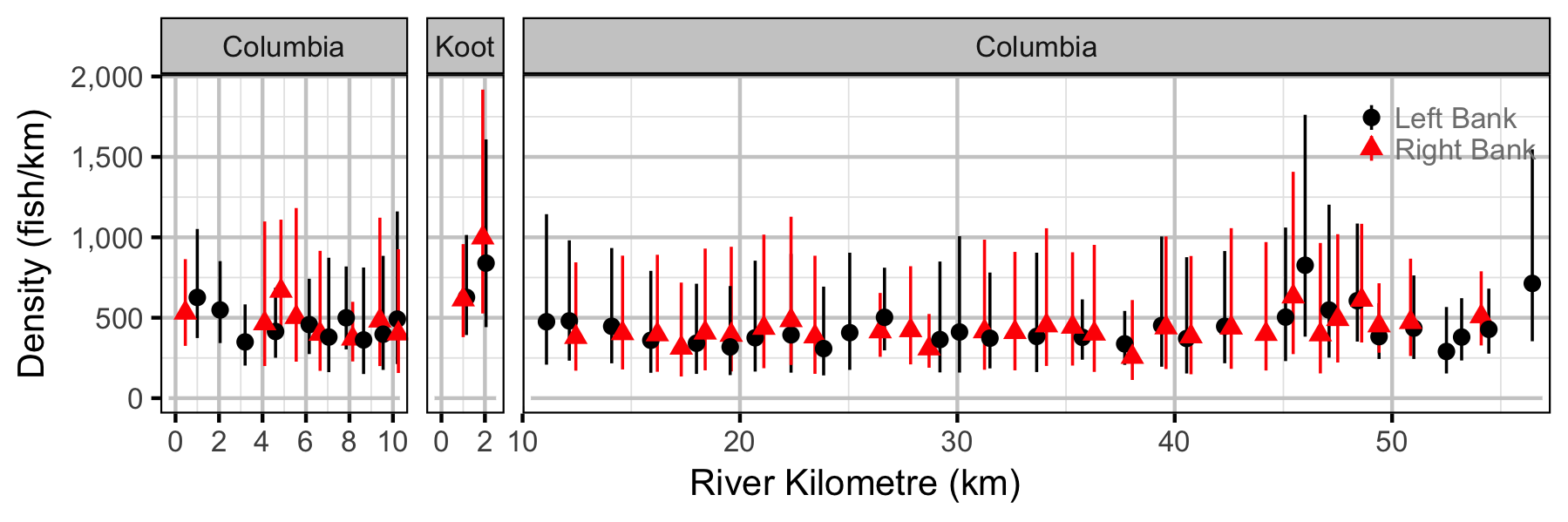Figure 42. Estimated lineal river count density of adult Walleye by site in (with 95% CIs).

### Stock-Recruitment

#### Mountain Whitefish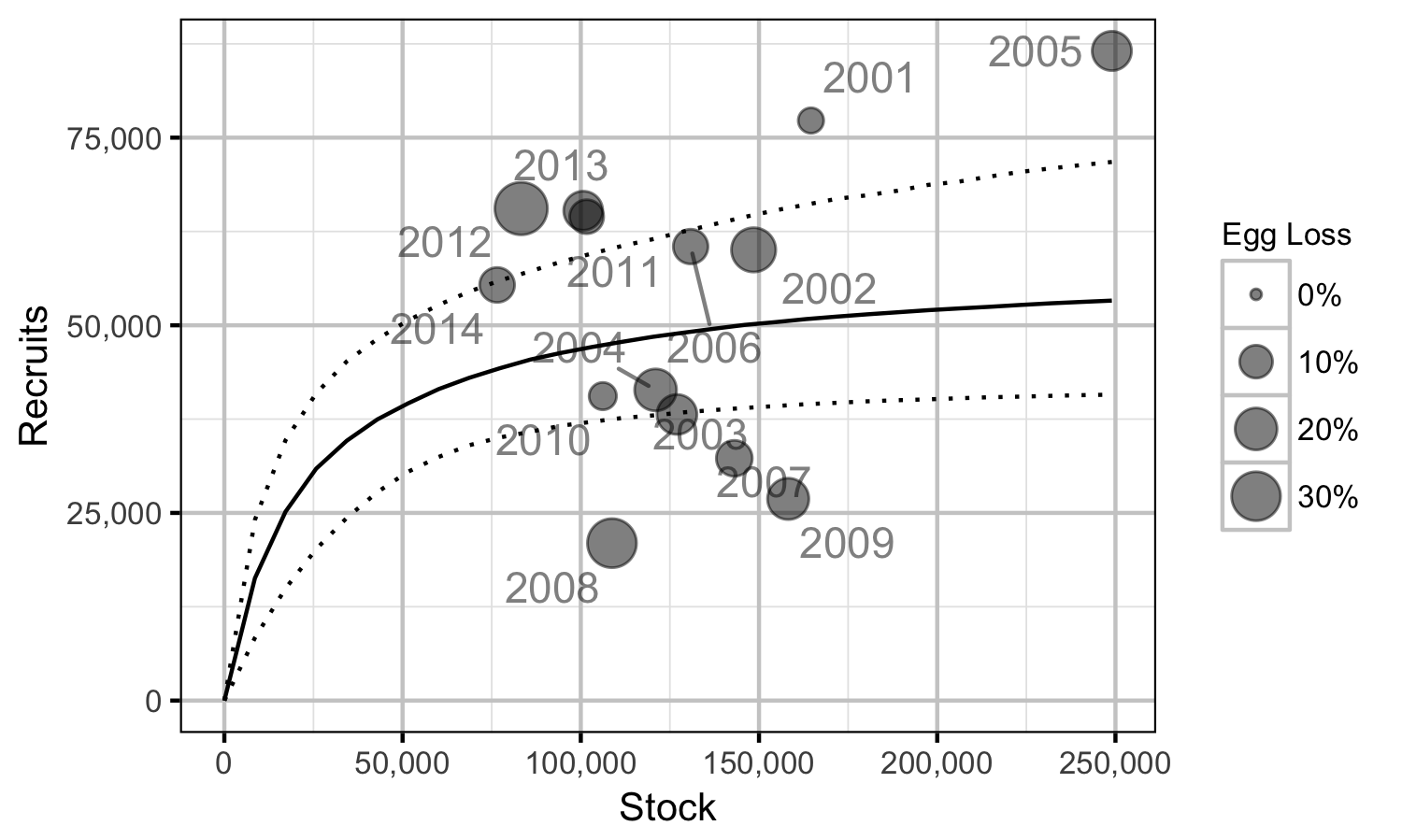Figure 43. Predicted stock-recruitment relationship from Age-2+ spawners to subsequent Age-1 recruits by spawn year (with 95% CRIs).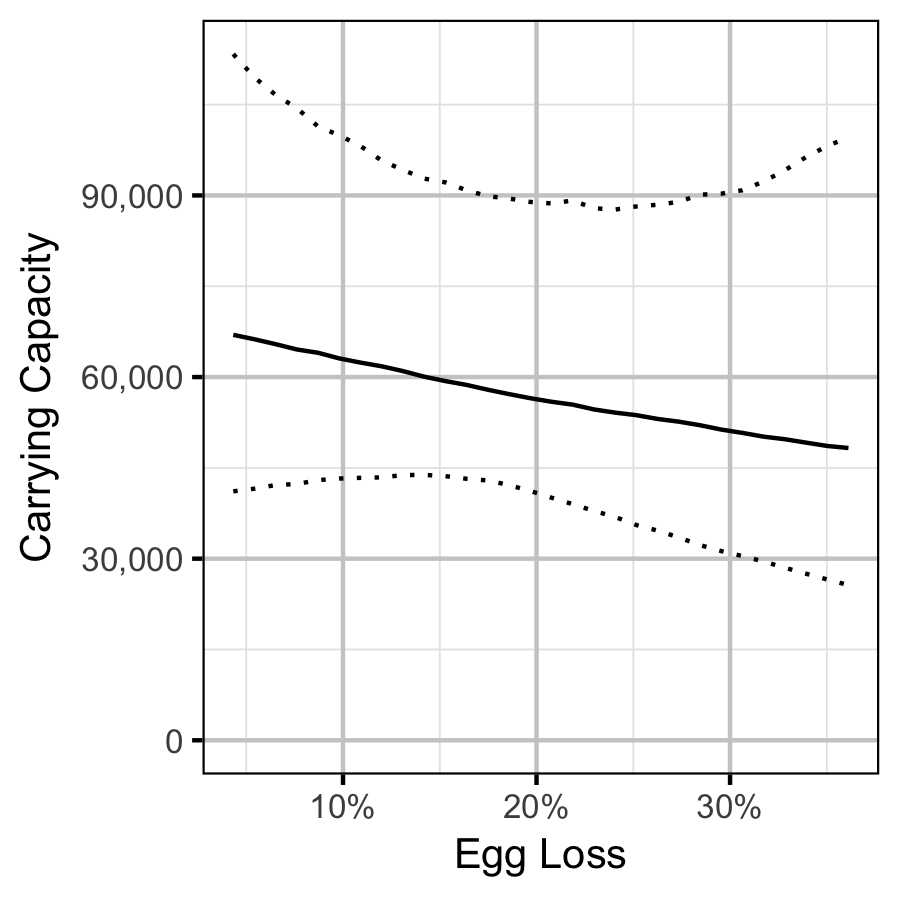Figure 44. Predicted Age-1 recruits carrying capacity by percentage egg loss (with 95% CRIs).

#### Rainbow Trout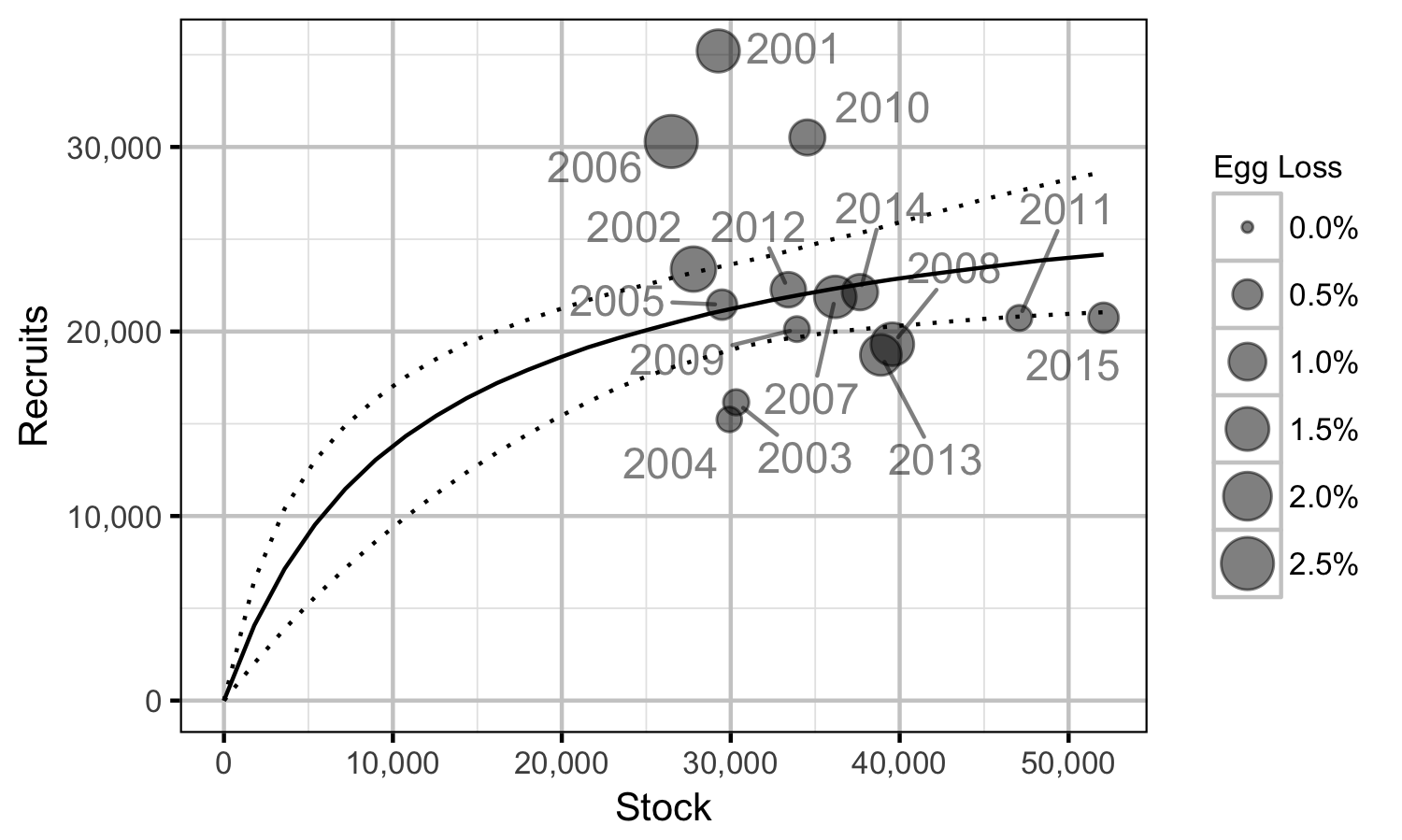Figure 45. Predicted stock-recruitment relationship from Age-2+ spawners to subsequent Age-1 recruits by spawn year (with 95% CRIs).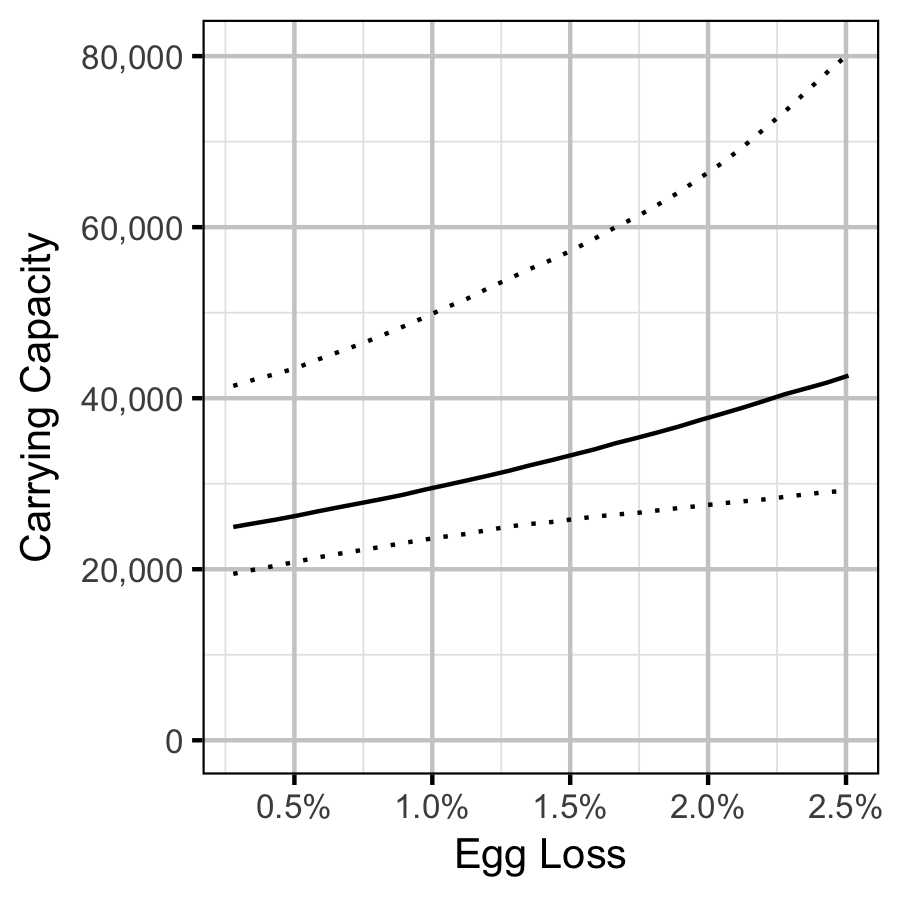Figure 46. Predicted Age-1 recruits carrying capacity by percentage egg loss (with 95% CRIs).

### Scale Age

#### Mountain Whitefish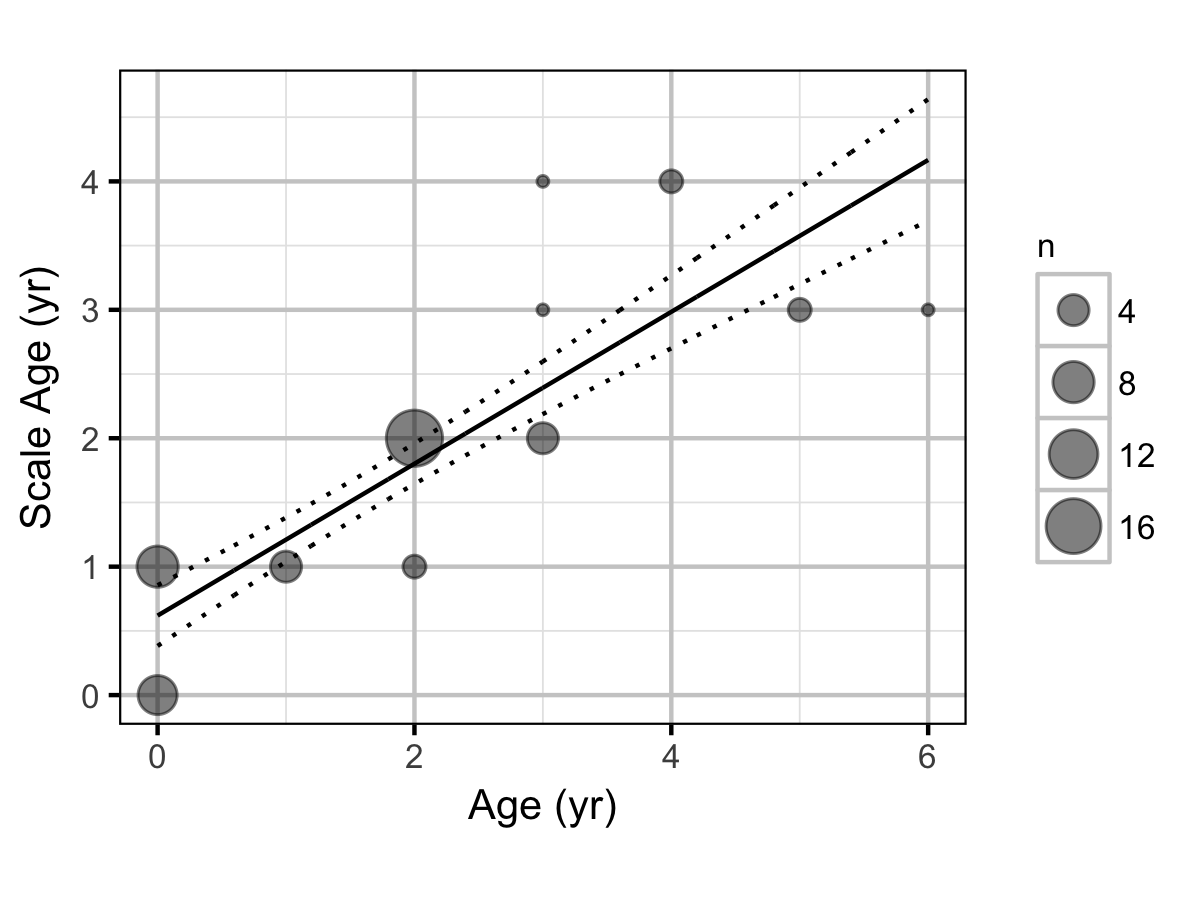Figure 47. Estimated scale age of Mountain Whitefish by actual age (with 95% CRIs). Scale ages were estimated using an automated algorithm.

### Age-Ratios

#### Mountain Whitefish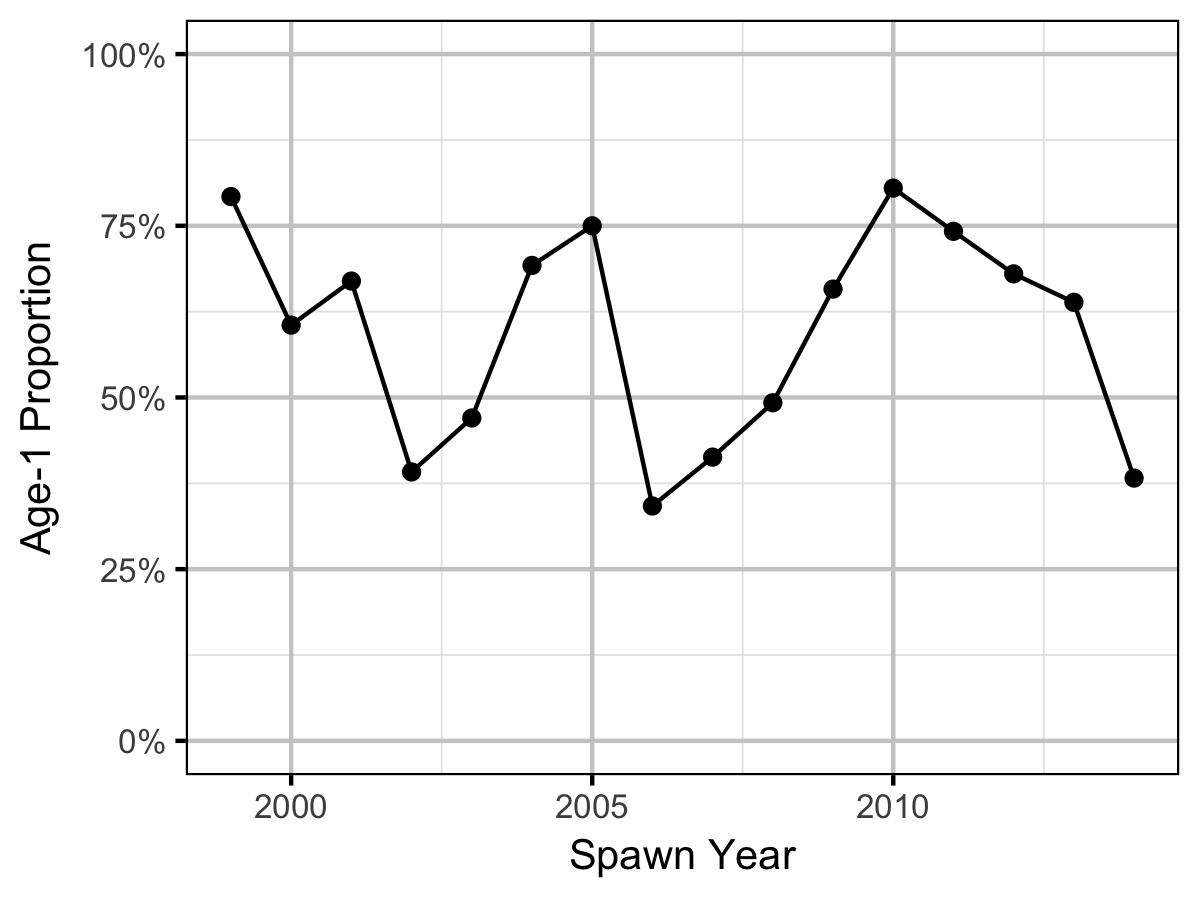Figure 48. Proportion of Age-1 Mountain Whitefish by spawn year.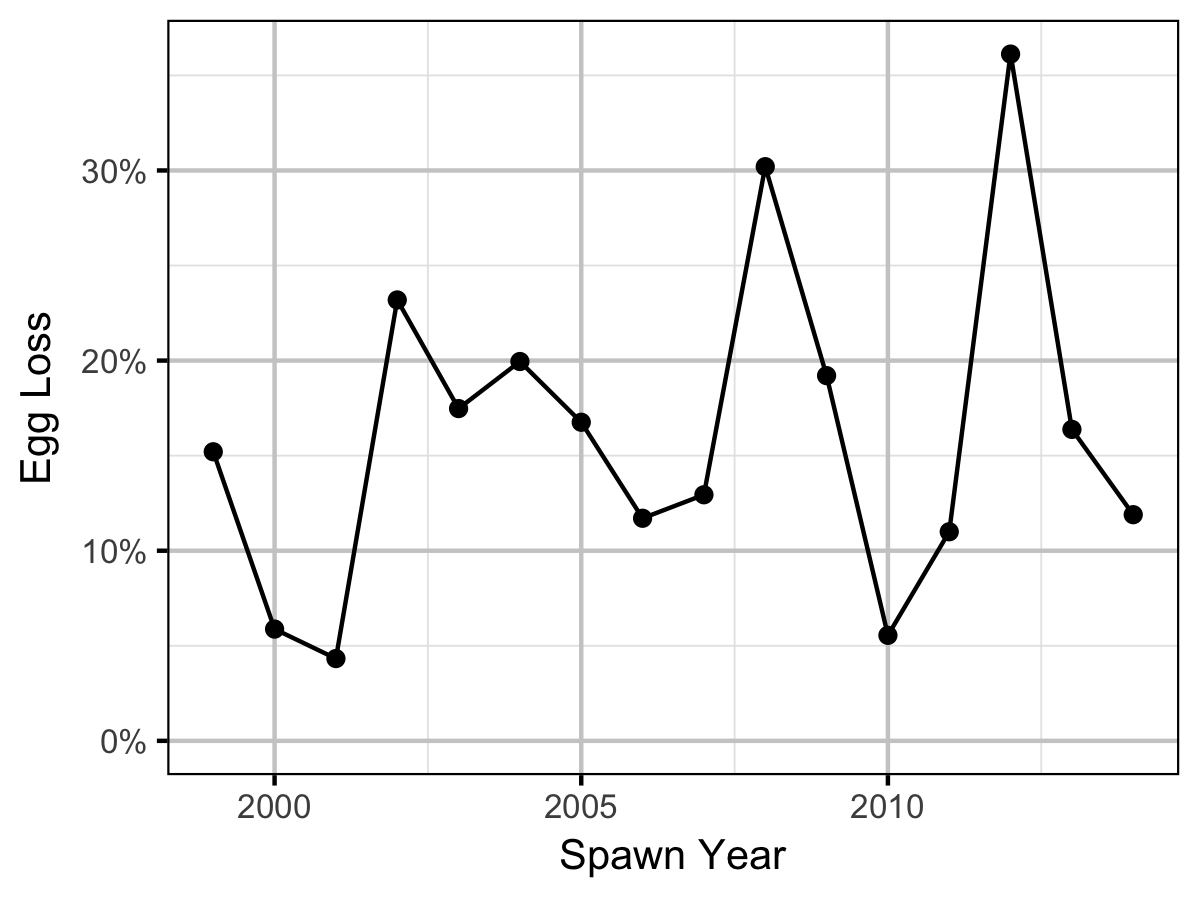Figure 49. Percentage Mountain Whitefish egg loss by spawn year.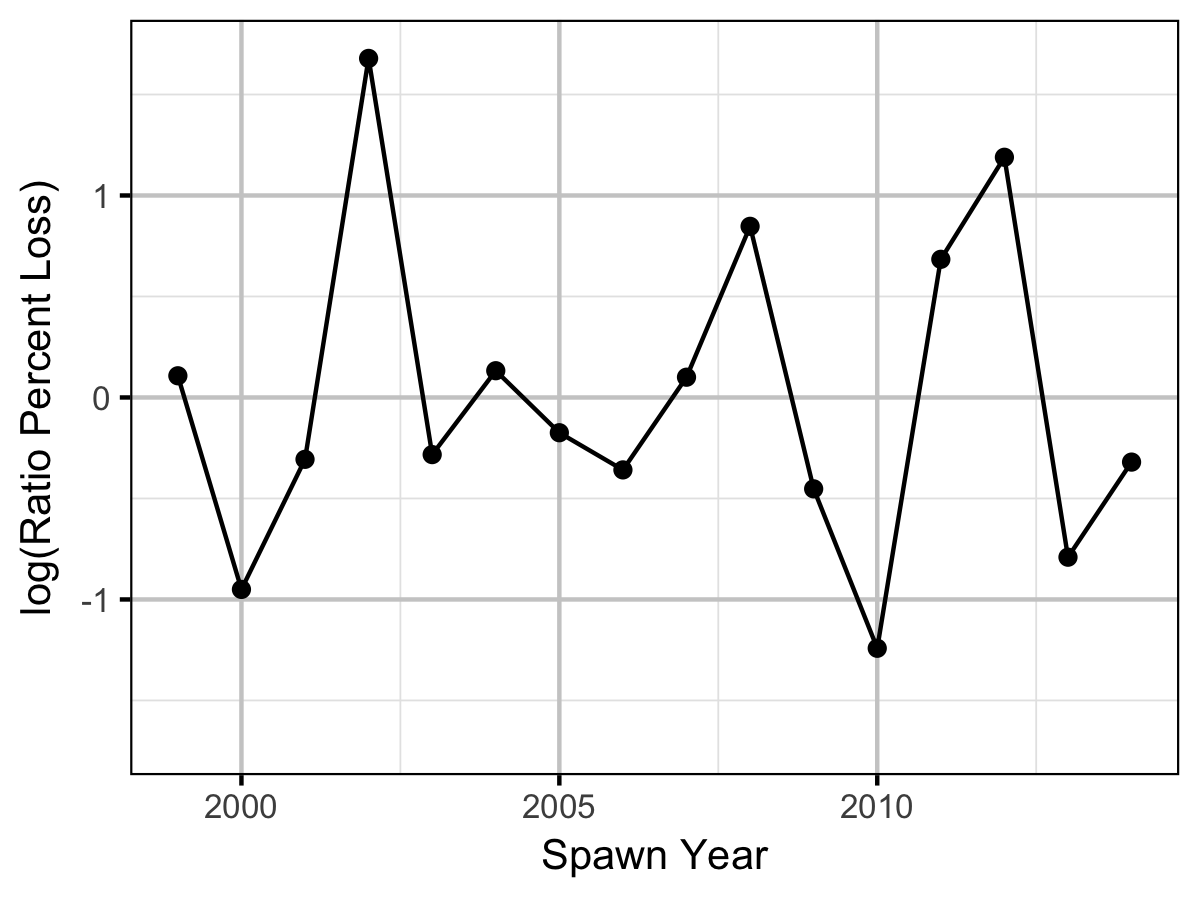Figure 50. Mountain Whitefish percentage egg loss ratio by spawn year.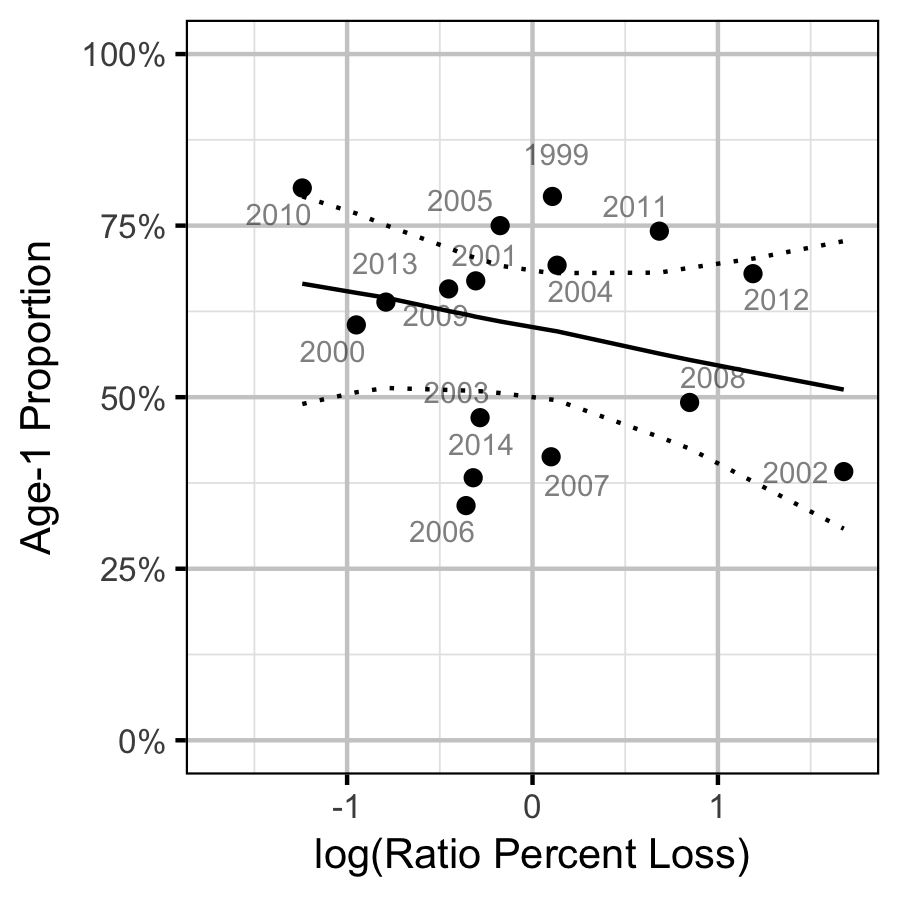Figure 51. Proportion of Age-1 Mountain Whitefish by percentage egg loss ratio, labelled by spawn year. The predicted relationship is indicated by the solid black line (with 95% CRIs).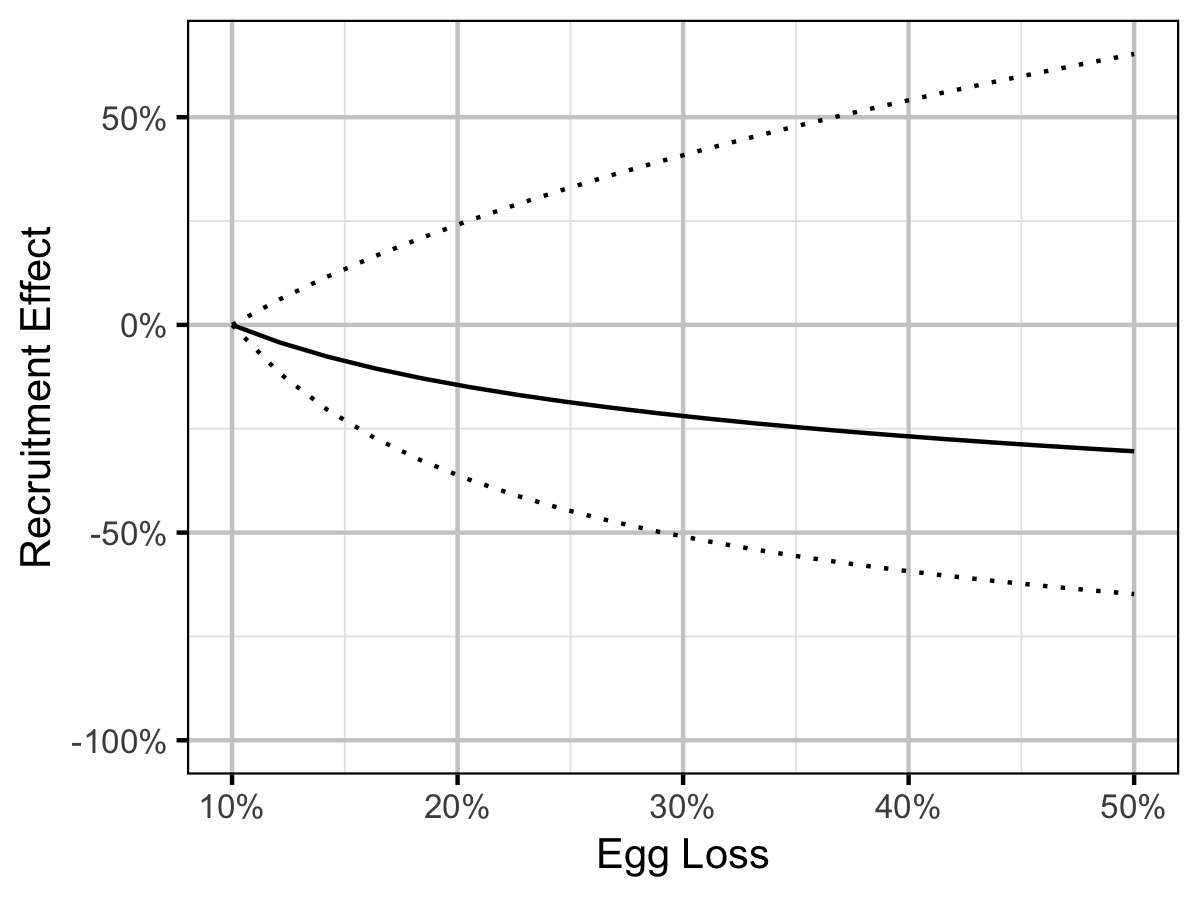Figure 52. Predicted percent change in Age-1 Mountain Whitefish abundance by egg loss in the spawn year relative to 10% egg loss in the spawn year (with 95% CRIs).

## Acknowledgements

The organisations and individuals whose contributions have made this analysis report possible include: